## Overview

ggrepel provides geoms for ggplot2 to repel overlapping text labels:

• geom_text_repel()
• geom_label_repel()

Text labels repel away from each other, away from data points, and away from edges of the plotting area (panel).

Let’s compare geom_text() and geom_text_repel():

library(ggrepel)
set.seed(42)

dat <- subset(mtcars, wt > 2.75 & wt < 3.45)
dat$car <- rownames(dat) p <- ggplot(dat, aes(wt, mpg, label = car)) + geom_point(color = "red") p1 <- p + geom_text() + labs(title = "geom_text()") p2 <- p + geom_text_repel() + labs(title = "geom_text_repel()") gridExtra::grid.arrange(p1, p2, ncol = 2)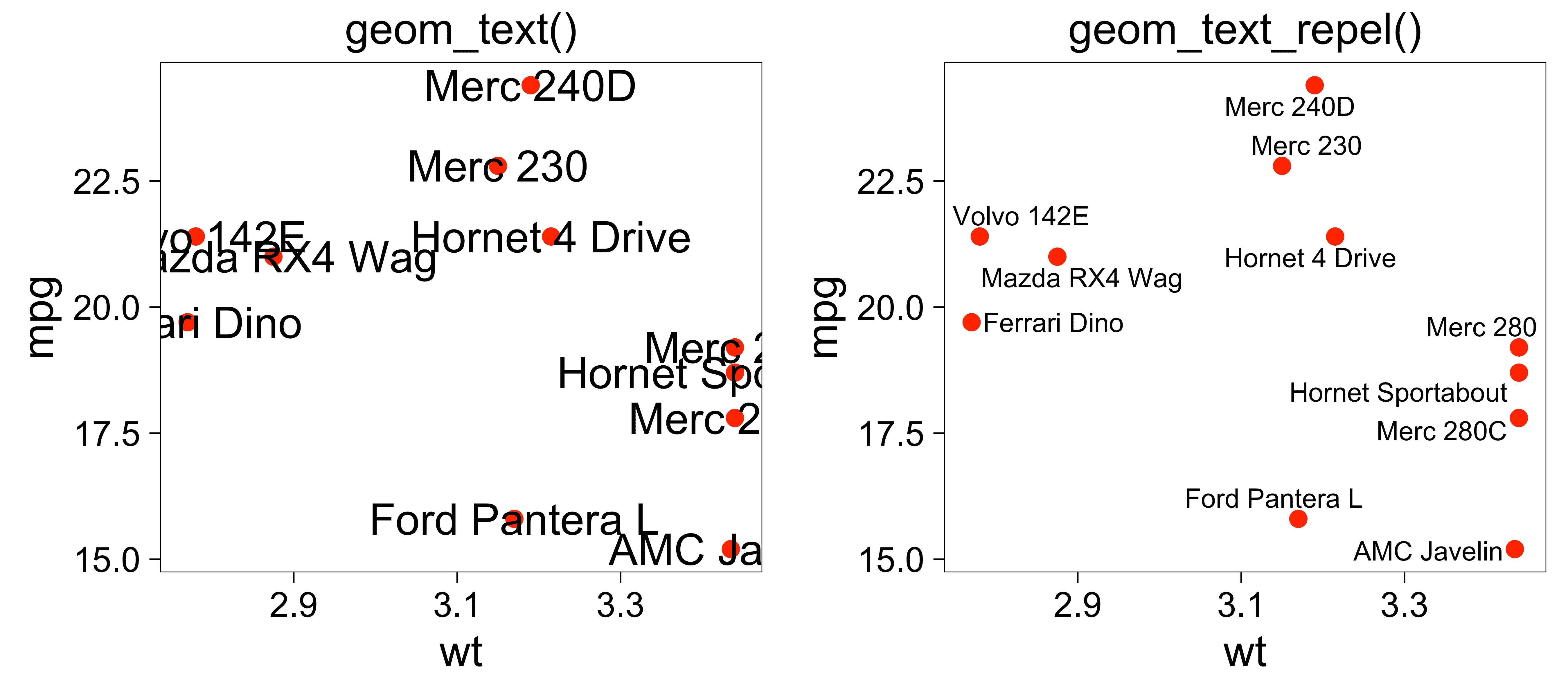## Installation ggrepel is available on CRAN: install.packages("ggrepel") The latest development version may have new features, and you can get it from GitHub: # Use the devtools package # install.packages("devtools") devtools::install_github("slowkow/ggrepel") ## Options Options allow us to change the behavior of ggrepel to fit the needs of our figure. Most of them are global options that affect all of the text labels, but some can be vectors of the same length as your data, like nudge_x or nudge_y. Option Default Description seed NA random seed for recreating the exact same layout force 1 force of repulsion between overlapping text labels force_pull 1 force of attraction between each text label and its data point direction "both" move text labels “both” (default), “x”, or “y” directions max.time 0.5 maximum number of seconds to try to resolve overlaps max.iter 10000 maximum number of iterations to try to resolve overlaps nudge_x 0 adjust the starting x position of the text label nudge_y 0 adjust the starting y position of the text label box.padding 0.25 lines padding around the text label point.padding 0 lines padding around the labeled data point arrow NULL render line segment as an arrow with grid::arrow() min.segment.length 0.5 only draw line segments that are longer than 0.5 (default) ## Aesthetics Aesthetics are parameters that can be mapped to your data with geom_text_repel(mapping = aes(...)). ggrepel provides the same aesthetics for geom_text_repel and geom_label_repel that are available in geom_text() or geom_label(), but it also provides a few more that are unique to ggrepel. All of them are listed below. See the ggplot2 documentation about aesthetic specifications for more details and examples. Aesthetic Default Description color "black" text and label border color size 3.88 font size angle 0 angle of the text label alpha NA transparency of the text label family "" font name fontface 1 “plain”, “bold”, “italic”, “bold.italic” lineheight 1.2 line height for text labels hjust 0.5 horizontal justification vjust 0.5 vertical justification point.size 1 size of each point for each text label segment.linetype 1 line segment solid, dashed, etc. segment.color "black" line segment color segment.size 0.5 mm line segment thickness segment.alpha 1.0 line segment transparency segment.curvature 0 numeric, negative for left-hand and positive for right-hand curves, 0 for straight lines segment.angle 90 0-180, less than 90 skews control points toward the start point segment.ncp 1 number of control points to make a smoother curve segment.shape 0.5 curve shape by control points approximation/interpolation (1 for cubic B-spline, -1 for Catmull-Rom spline) segment.square TRUE TRUE to place control points in city-block fashion, FALSE for oblique placement segment.squareShape 1 shape of the curve relative to additional control points inserted if square is TRUE segment.inflect FALSE curve inflection at the midpoint segment.debug FALSE display the curve debug information ## Examples ### Hide some of the labels Set labels to the empty string "" to hide them. All data points repel the non-empty labels. set.seed(42) dat2 <- subset(mtcars, wt > 3 & wt < 4) # Hide all of the text labels. dat2$car <- ""
# Let's just label these items.
ix_label <- c(2, 3, 14)
dat2$car[ix_label] <- rownames(dat2)[ix_label] ggplot(dat2, aes(wt, mpg, label = car)) + geom_text_repel() + geom_point(color = ifelse(dat2$car == "", "grey50", "red"))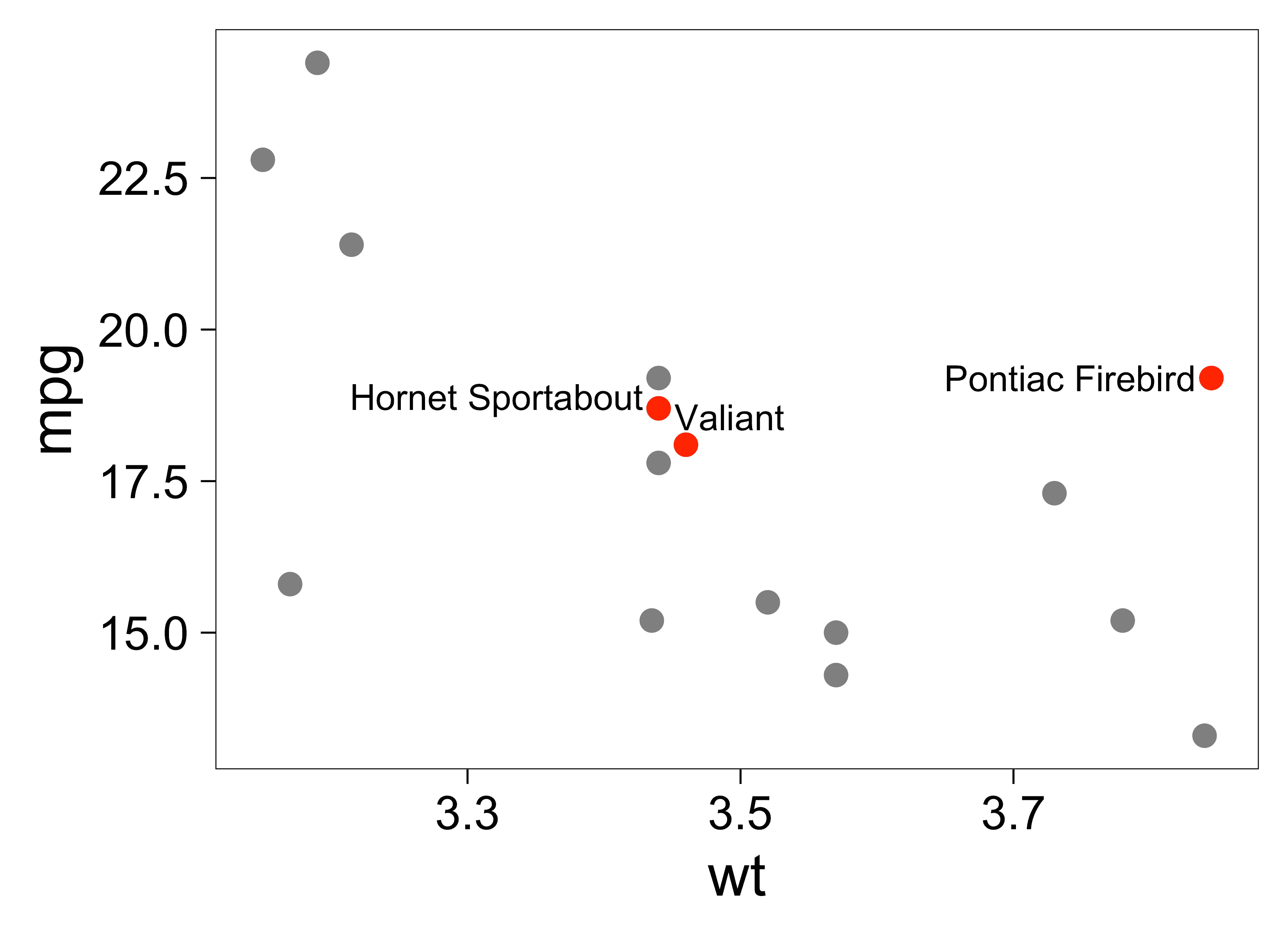We can quickly repel a few text labels from 10,000 data points:

set.seed(42)

dat3 <- rbind(
data.frame(
wt  = rnorm(n = 10000, mean = 3),
mpg = rnorm(n = 10000, mean = 19),
car = ""
),
dat2[,c("wt", "mpg", "car")]
)

ggplot(dat3, aes(wt, mpg, label = car)) +
geom_point(data = dat3[dat3$car == "",], color = "grey50") + geom_text_repel(box.padding = 0.5, max.overlaps = Inf) + geom_point(data = dat3[dat3$car != "",], color = "red")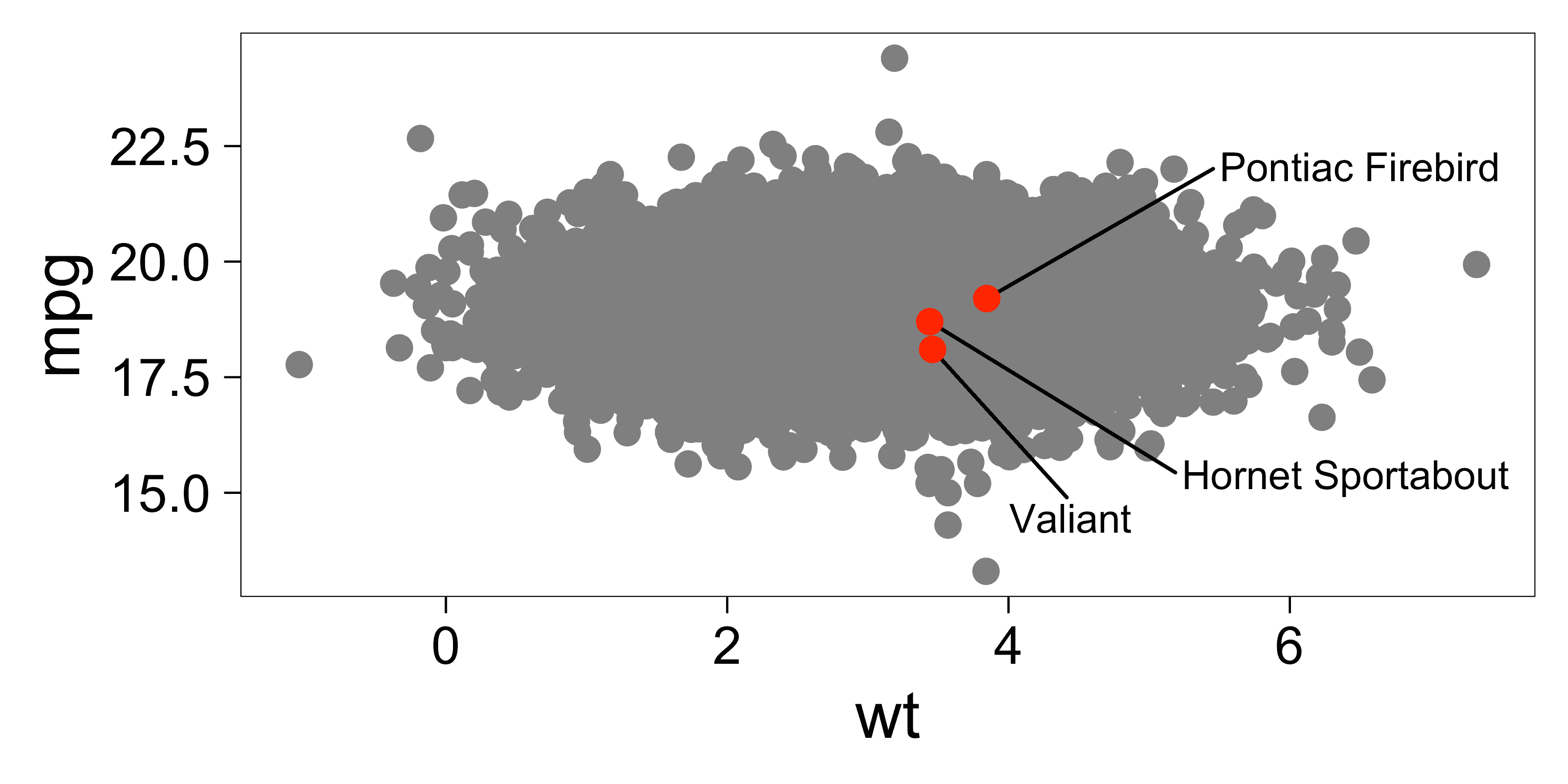### Always show all labels, even when they have too many overlaps

Some text labels will be discarded if they have too many overlaps.

We can expect to see a warning if some data points could not be labeled due to too many overlaps.

Set max.overlaps = Inf to override this behavior and always show all labels, regardless of too many overlaps.

Use options(ggrepel.max.overlaps = Inf) to set this globally for your entire session. The global option can be overridden by providing the max.overlaps argument to geom_text_repel().

set.seed(42)

n <- 15
dat4 <- data.frame(
x = rep(1, length.out = n),
y = rep(1, length.out = n),
label = letters[1:n]
)

# Set it globally:
options(ggrepel.max.overlaps = Inf)

p1 <- ggplot(dat4, aes(x, y, label = label)) +
geom_point() +
geom_label_repel(box.padding = 0.5, max.overlaps = 10) +
labs(title = "max.overlaps = 10 (default)")

p2 <- ggplot(dat4, aes(x, y, label = label)) +
geom_point() +
labs(title = "max.overlaps = Inf")

gridExtra::grid.arrange(p1, p2, ncol = 2)
## Warning: ggrepel: 15 unlabeled data points (too many overlaps). Consider
## increasing max.overlaps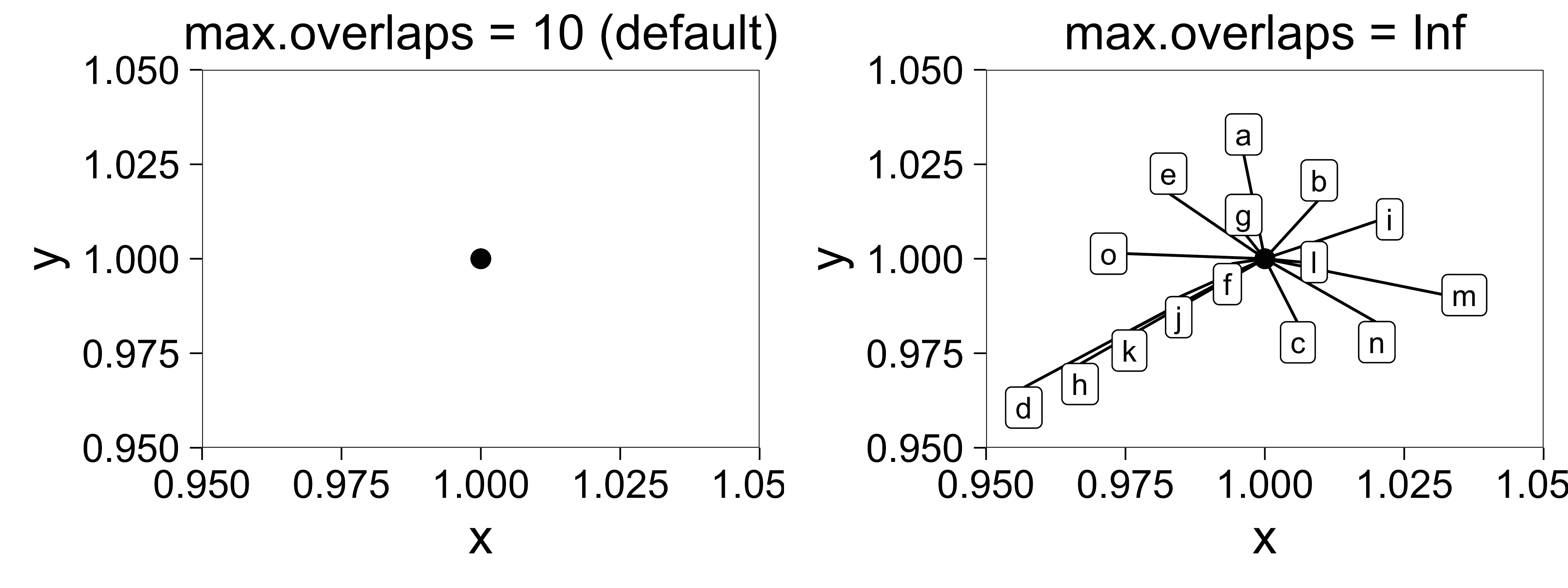### Do not repel labels from data points

Set point.size = NA to prevent label repulsion away from data points.

Labels will still move away from each other and away from the edges of the plot.

set.seed(42)
ggplot(dat, aes(wt, mpg, label = car)) +
geom_point(color = "red") +
geom_text_repel(point.size = NA)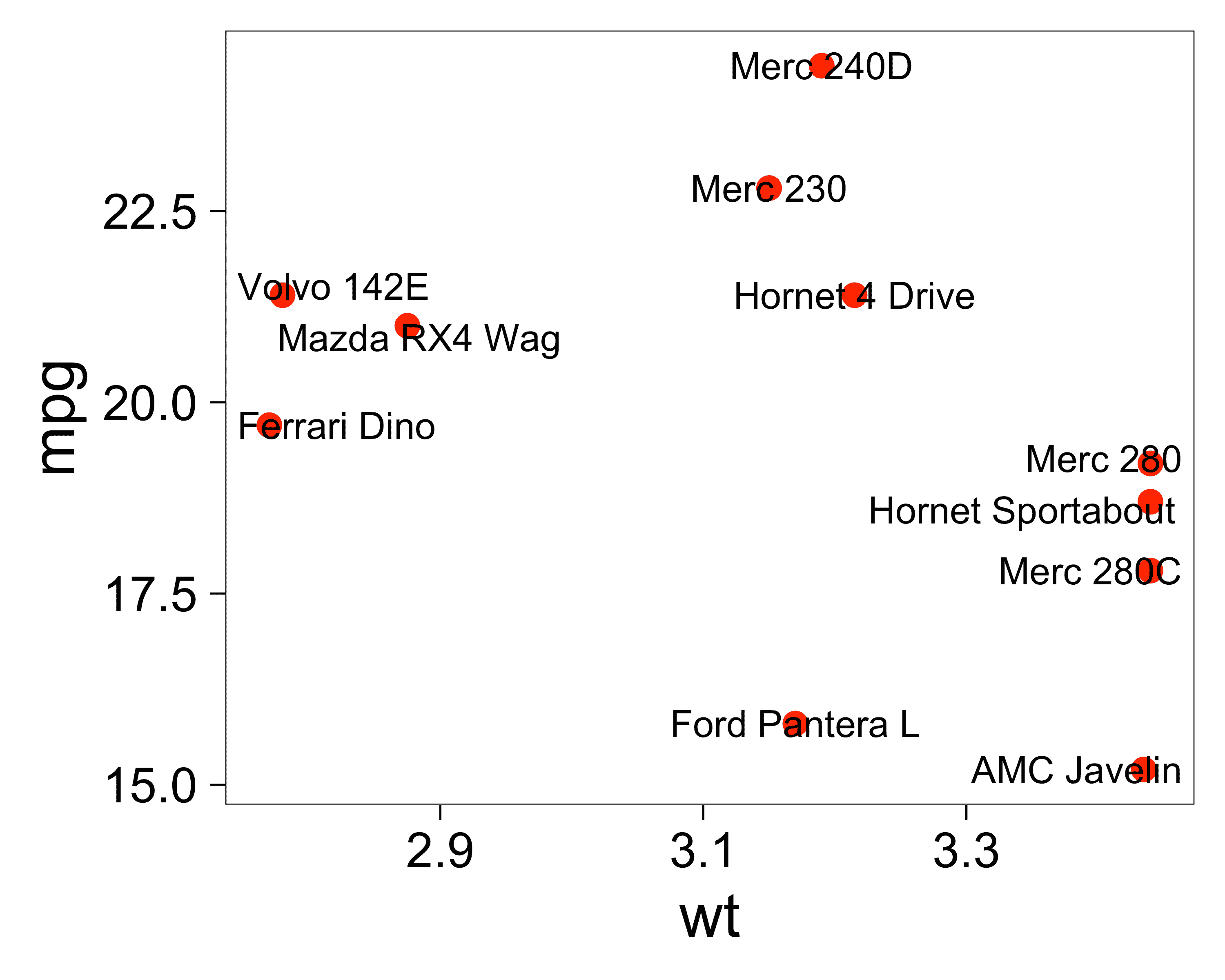### Do not repel labels from plot (panel) edges

Set xlim or ylim to Inf or -Inf to disable repulsion away from the edges of the panel. Use NA to indicate the edge of the panel.

set.seed(42)
ggplot(dat, aes(wt, mpg, label = car)) +
geom_point(color = "red") +
geom_text_repel(
# Repel away from the left edge, not from the right.
xlim = c(NA, Inf),
# Do not repel from top or bottom edges.
ylim = c(-Inf, Inf)
)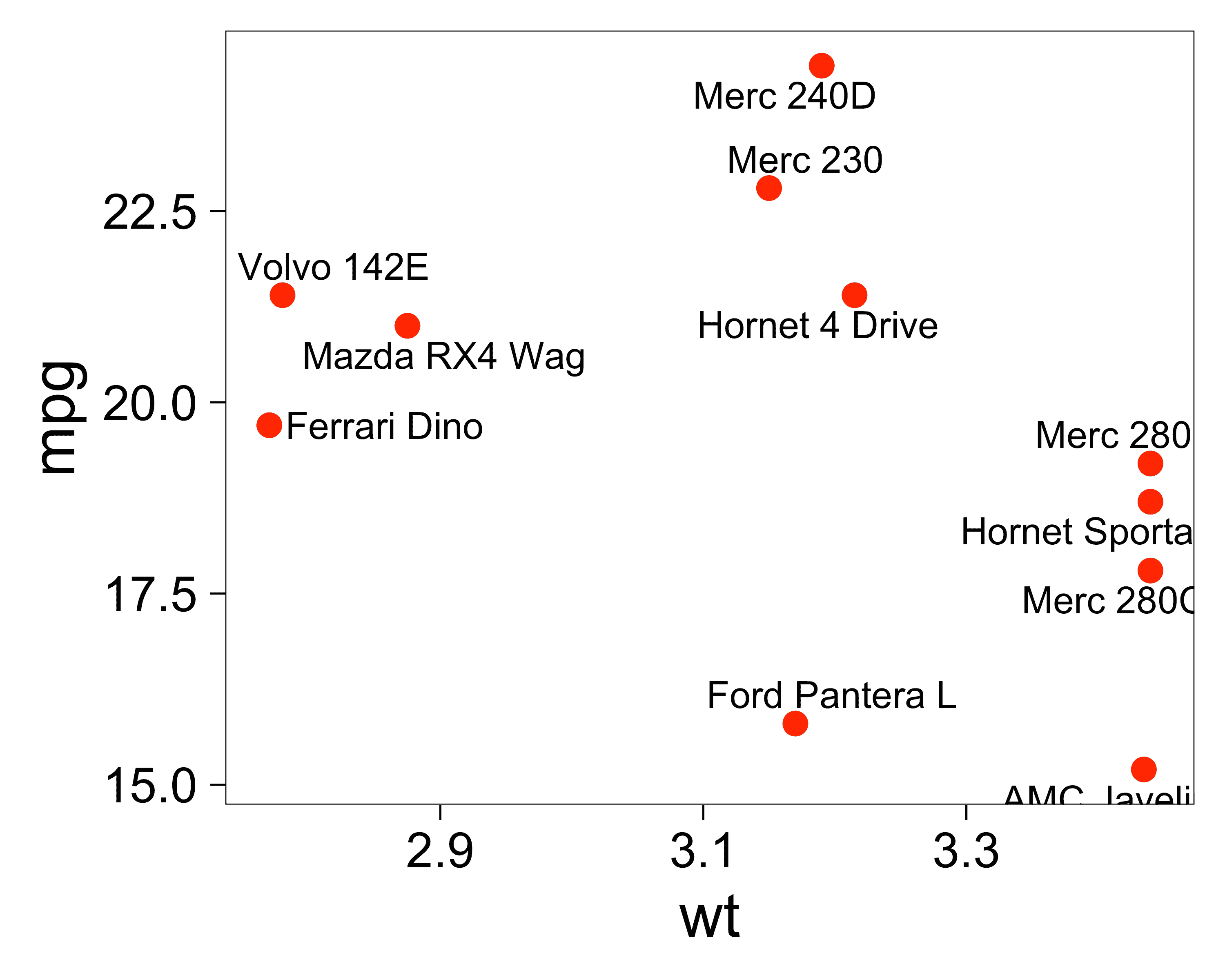We can also disable clipping to allow the labels to go beyond the edges of the panel.

set.seed(42)
ggplot(dat, aes(wt, mpg, label = car)) +
geom_point(color = "red") +
coord_cartesian(clip = "off") +
geom_label_repel(fill = "white", xlim = c(-Inf, Inf), ylim = c(-Inf, Inf))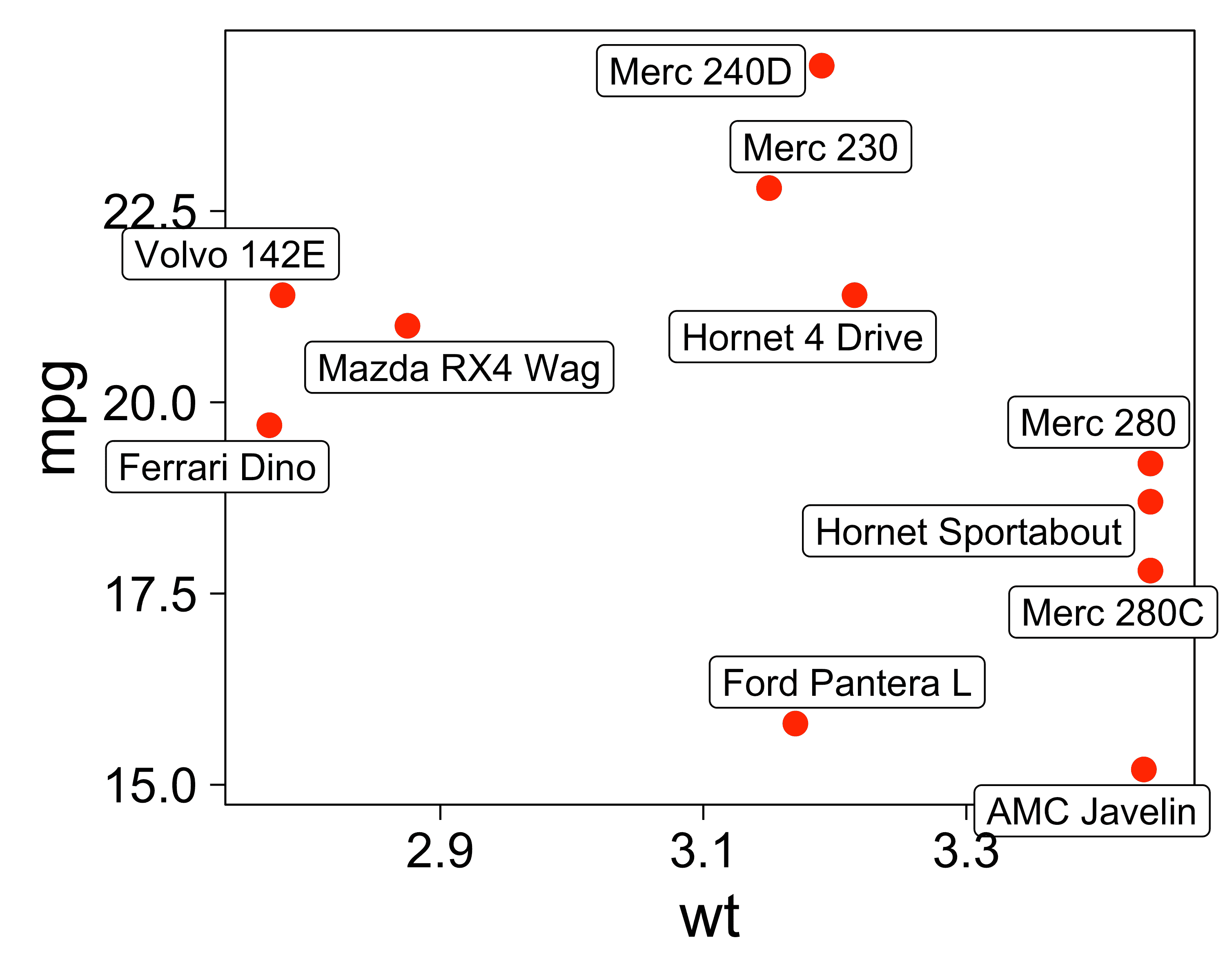### Expand the scale to make room for labels

Since the text labels repel away from the edges of the plot panel, we might want to expand the scale to give them more room to fit.

set.seed(42)
d <- data.frame(
x1 = 1,
y1 = rnorm(10),
x2 = 2,
y2 = rnorm(10),
lab = state.name[1:10]
)

p <- ggplot(d, aes(x1, y1, xend = x2, yend = y2, label = lab, col = lab)) +
geom_segment(size = 1) +
guides(color = "none") +
theme(axis.title.x = element_blank()) +
geom_text_repel(
nudge_x = -0.2, direction = "y", hjust = "right"
) +
geom_text_repel(
aes(x2, y2), nudge_x = 0.1, direction = "y", hjust = "left"
)

p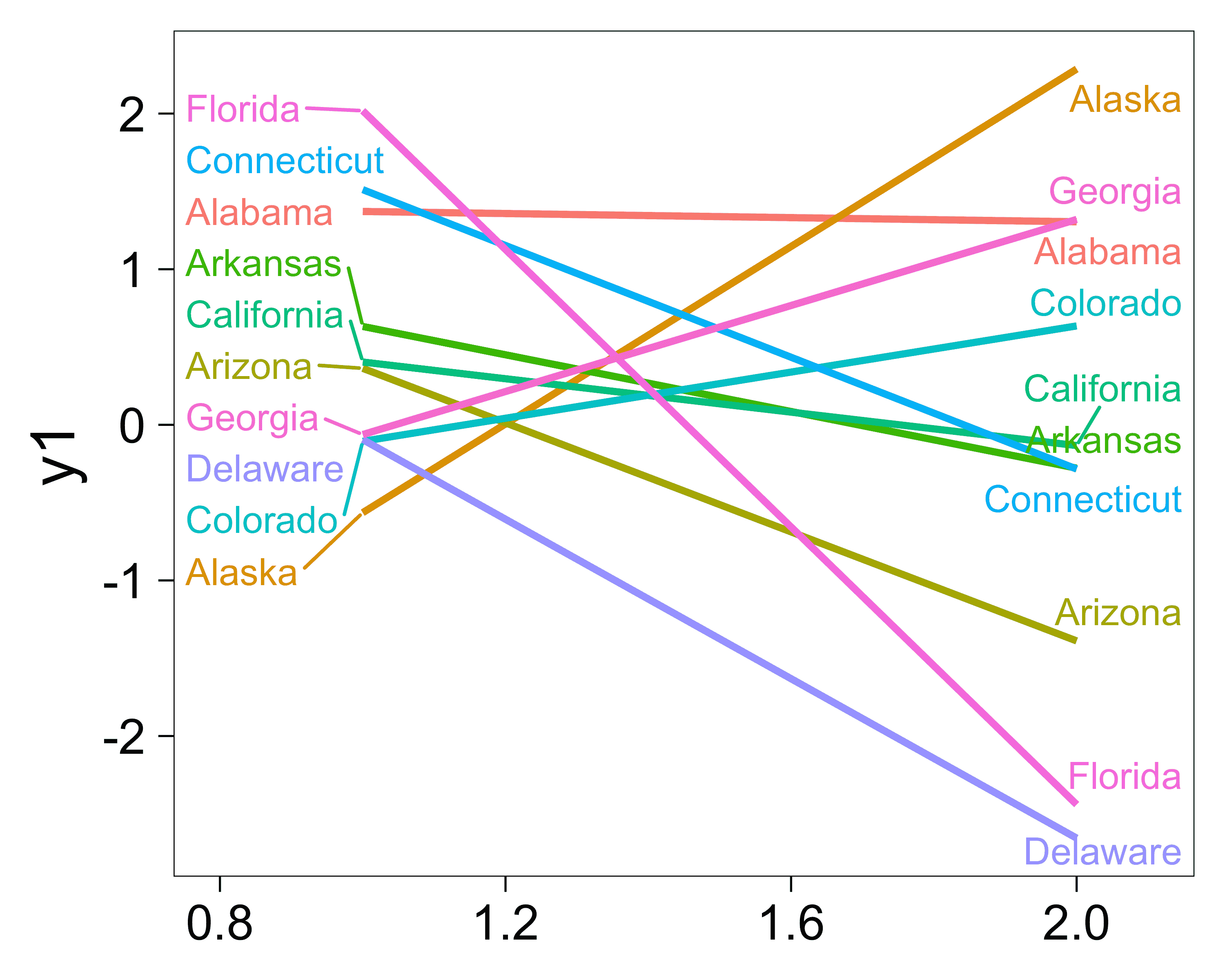p + scale_x_continuous(
breaks = 1:2, labels = c("Dimension 1", "Dimension 2"),
expand = expansion(mult = 0.5)
)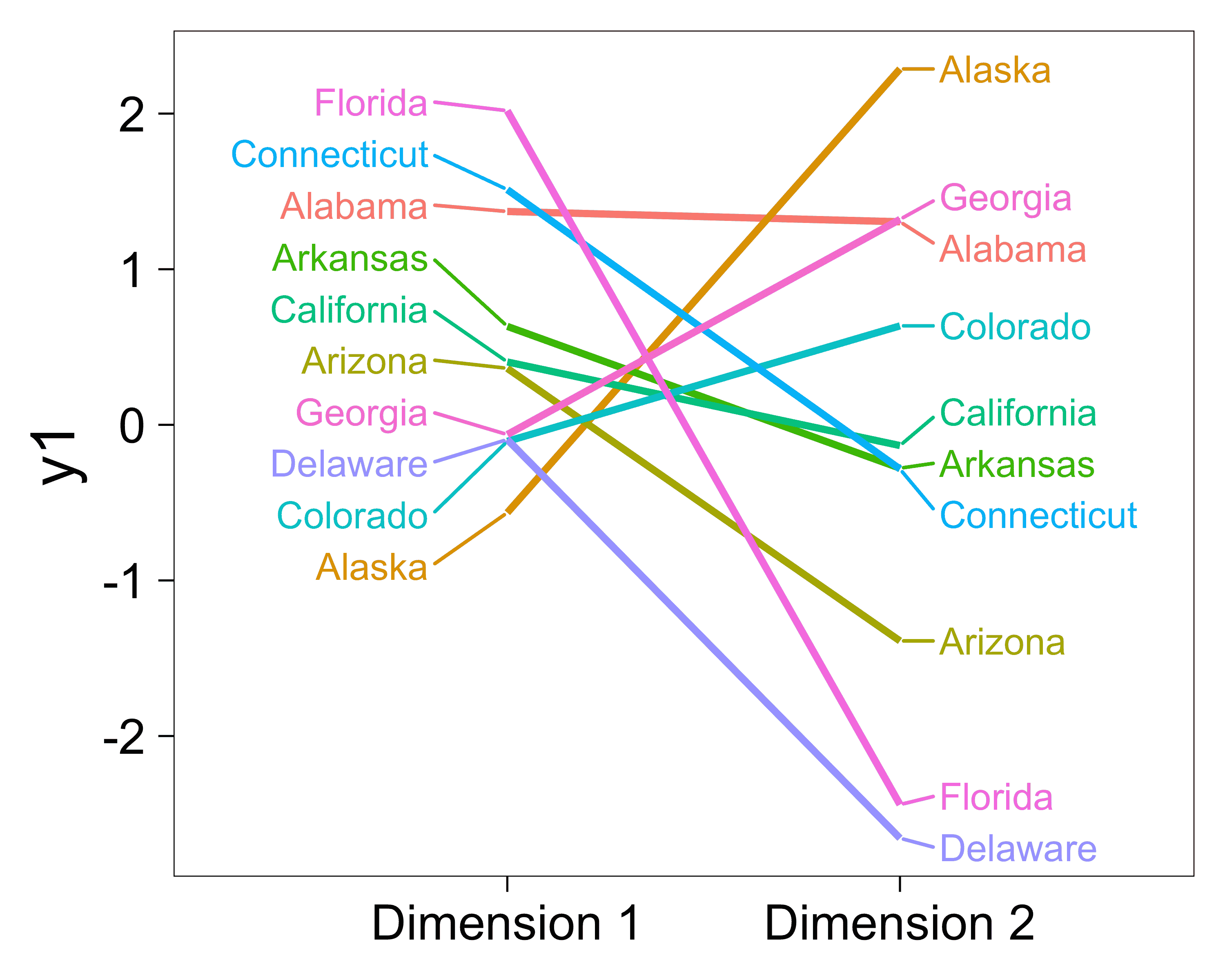### Always (or never) draw line segments

Use min.segment.length = 0 to draw all line segments, no matter how short they are.

Use min.segment.length = Inf to never draw any line segments, no matter how long they are.

p <- ggplot(dat, aes(wt, mpg, label = car)) +
geom_point(color = "red")

p1 <- p +
geom_text_repel(min.segment.length = 0, seed = 42, box.padding = 0.5) +
labs(title = "min.segment.length = 0")

p2 <- p +
geom_text_repel(min.segment.length = Inf, seed = 42, box.padding = 0.5) +
labs(title = "min.segment.length = Inf")

gridExtra::grid.arrange(p1, p2, ncol = 2)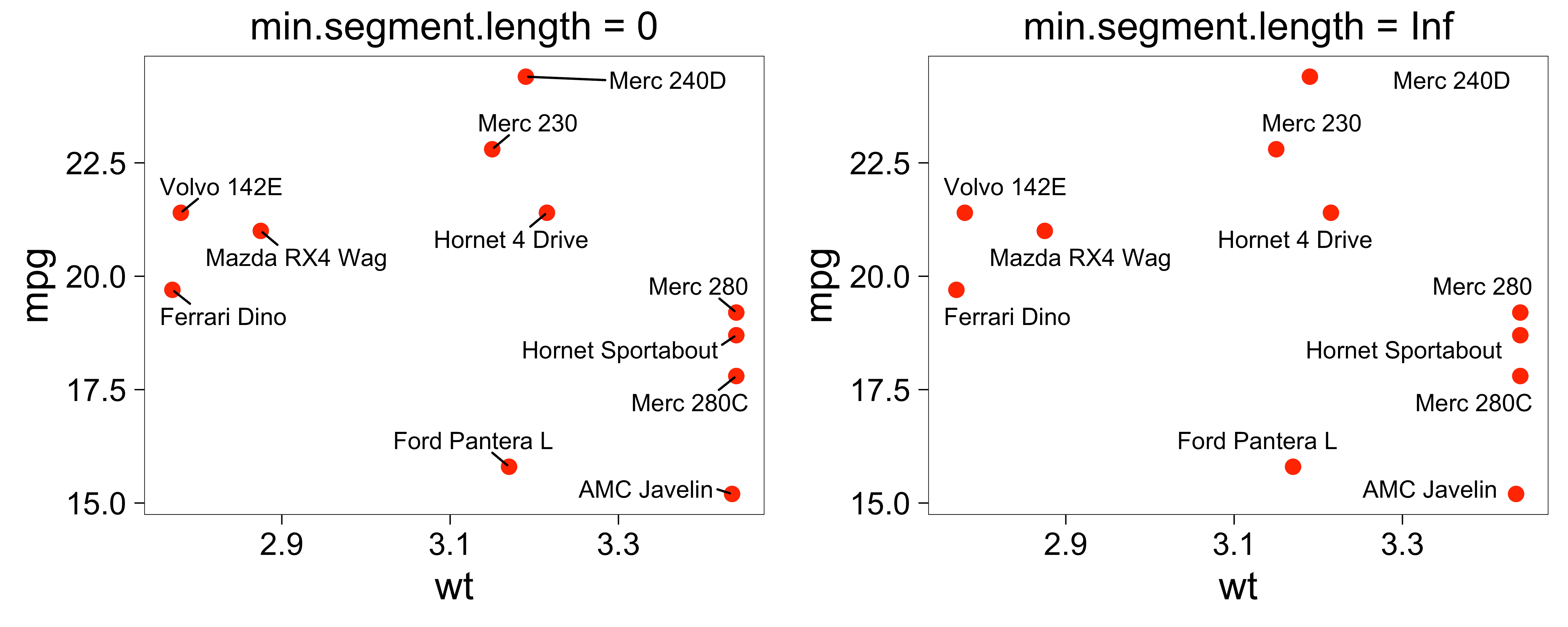### Make curved line segments or arrows

The line segments can be curved as in geom_curve() from ggplot2.

• segment.curvature = 1 increases right-hand curvature, negative values would increase left-hand curvature, 0 makes straight lines
• segment.ncp = 3 gives 3 control points for the curve
• segment.angle = 20 skews the curve towards the start, values greater than 90 would skew toward the end
set.seed(42)
ggplot(dat, aes(wt, mpg, label = car)) +
geom_point(color = "red") +
geom_text_repel(
nudge_x = .15,
nudge_y = 1,
segment.curvature = -0.1,
segment.ncp = 3,
segment.angle = 20
)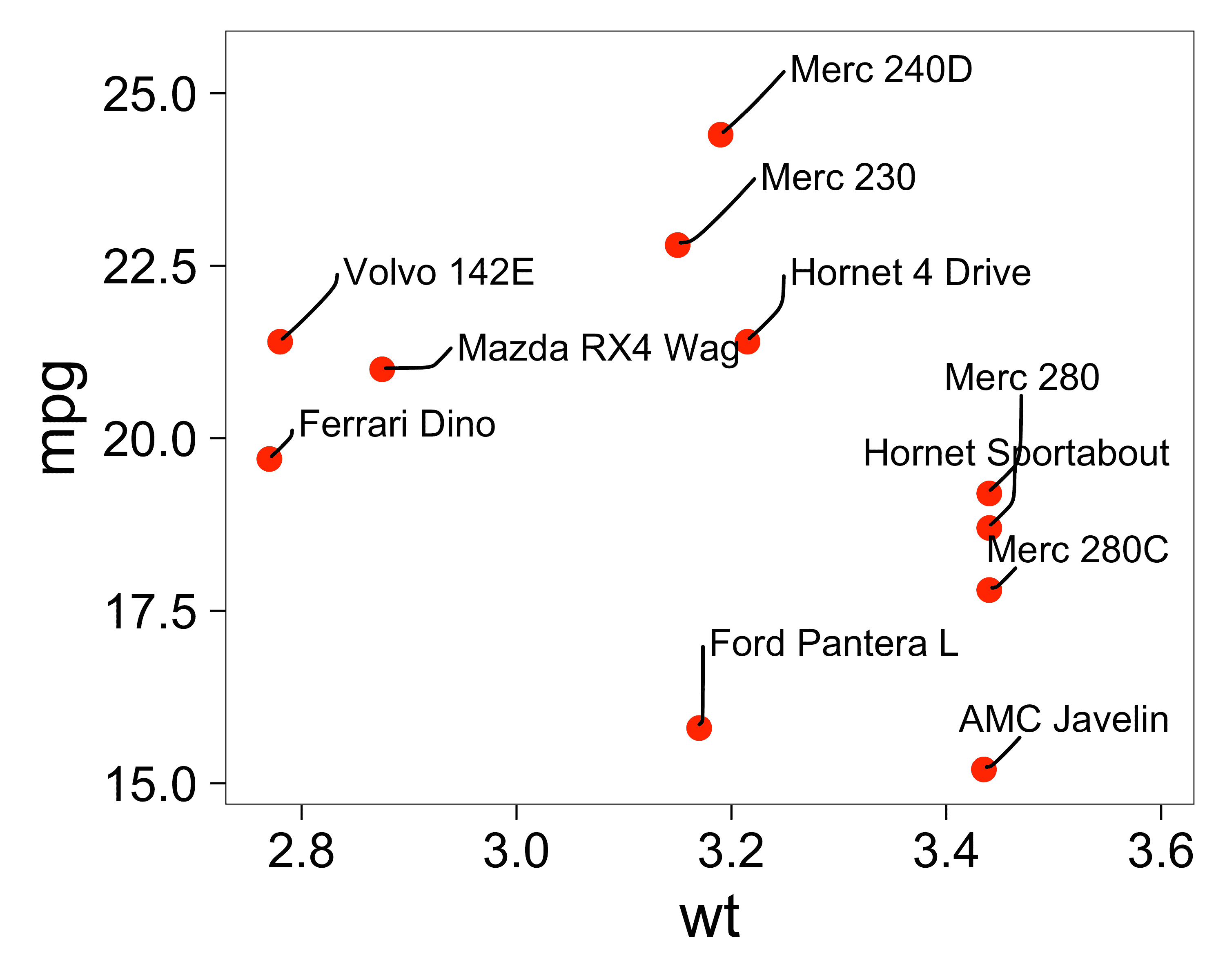Setting the curvature to a value near zero gives a sharp angle:

set.seed(42)
cars <- c("Volvo 142E", "Merc 230")

ggplot(dat) +
aes(wt, mpg, label = ifelse(car %in% cars, car, "")) +
geom_point(color = "red") +
geom_text_repel(
nudge_x = .15,
nudge_y = .5,
segment.curvature = -1e-20,
arrow = arrow(length = unit(0.015, "npc"))
) +
theme(legend.position = "none")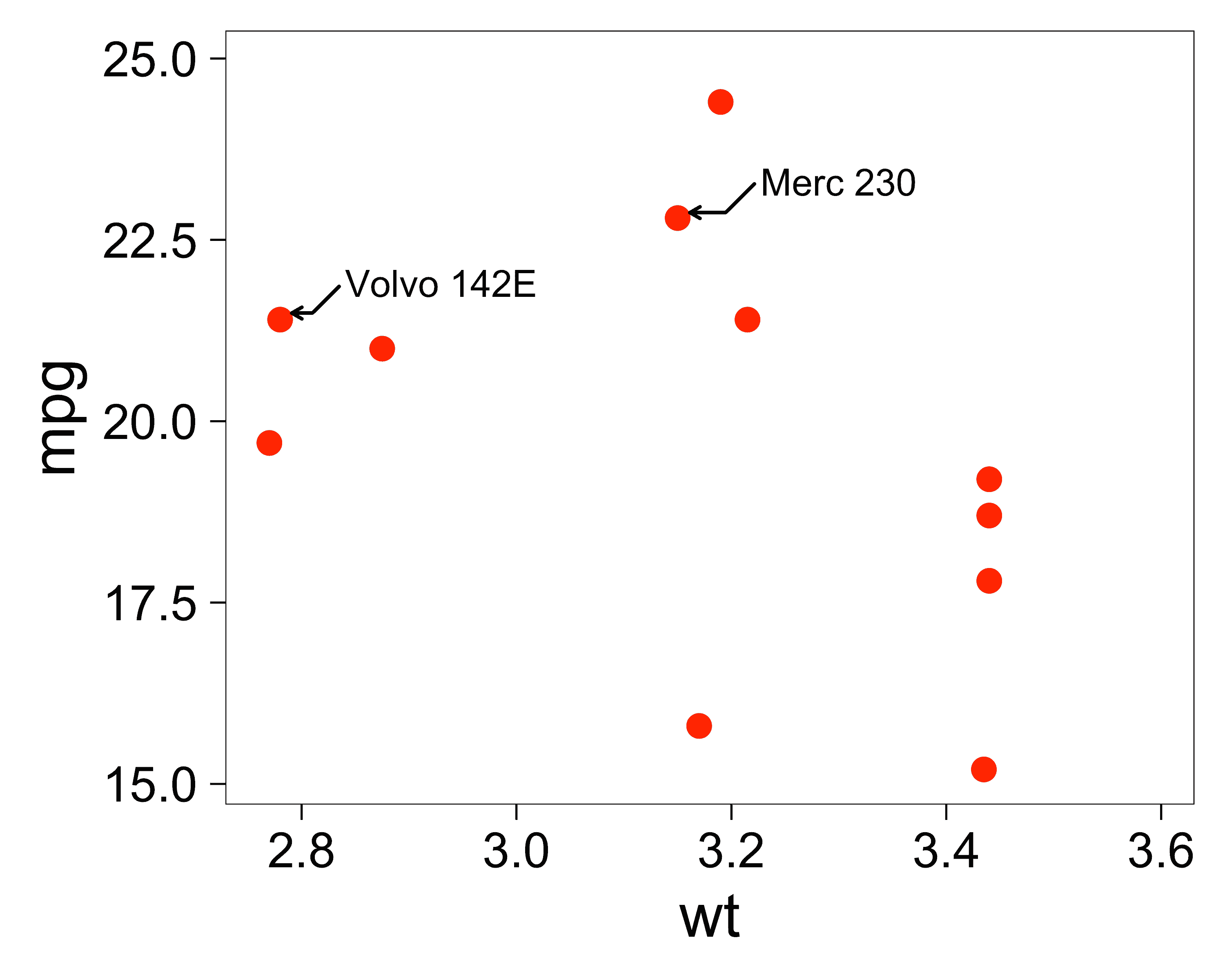Set segment.squareto FALSE to get oblique curves, and segment.inflect to TRUE to introduce an inflection point.

set.seed(42)

cars_subset$car <- rownames(cars_subset) cars_subset_curves <- cars_subset[rep(seq_len(nrow(cars_subset)), times = 4), ] cars_subset_curves$square <- rep(c(TRUE, FALSE), each = nrow(cars_subset) * 2)
cars_subset_curves$inflect <- rep(c(TRUE, FALSE, TRUE, FALSE), each = nrow(cars_subset)) ggplot(cars_subset_curves, aes(y = wt, x = 1, label = car)) + facet_grid(square ~ inflect, labeller = labeller(.default = label_both)) + geom_point(color = "red") + ylim(1, 4.5) + xlim(1, 1.375) + geom_text_repel( aes( segment.square = square, segment.inflect = inflect, ), force = 0.5, nudge_x = 0.15, direction = "y", hjust = 0, segment.size = 0.2, segment.curvature = -0.1 ) + theme( axis.line.x = element_blank(), axis.ticks.x = element_blank(), axis.text.x = element_blank(), axis.title.x = element_blank() )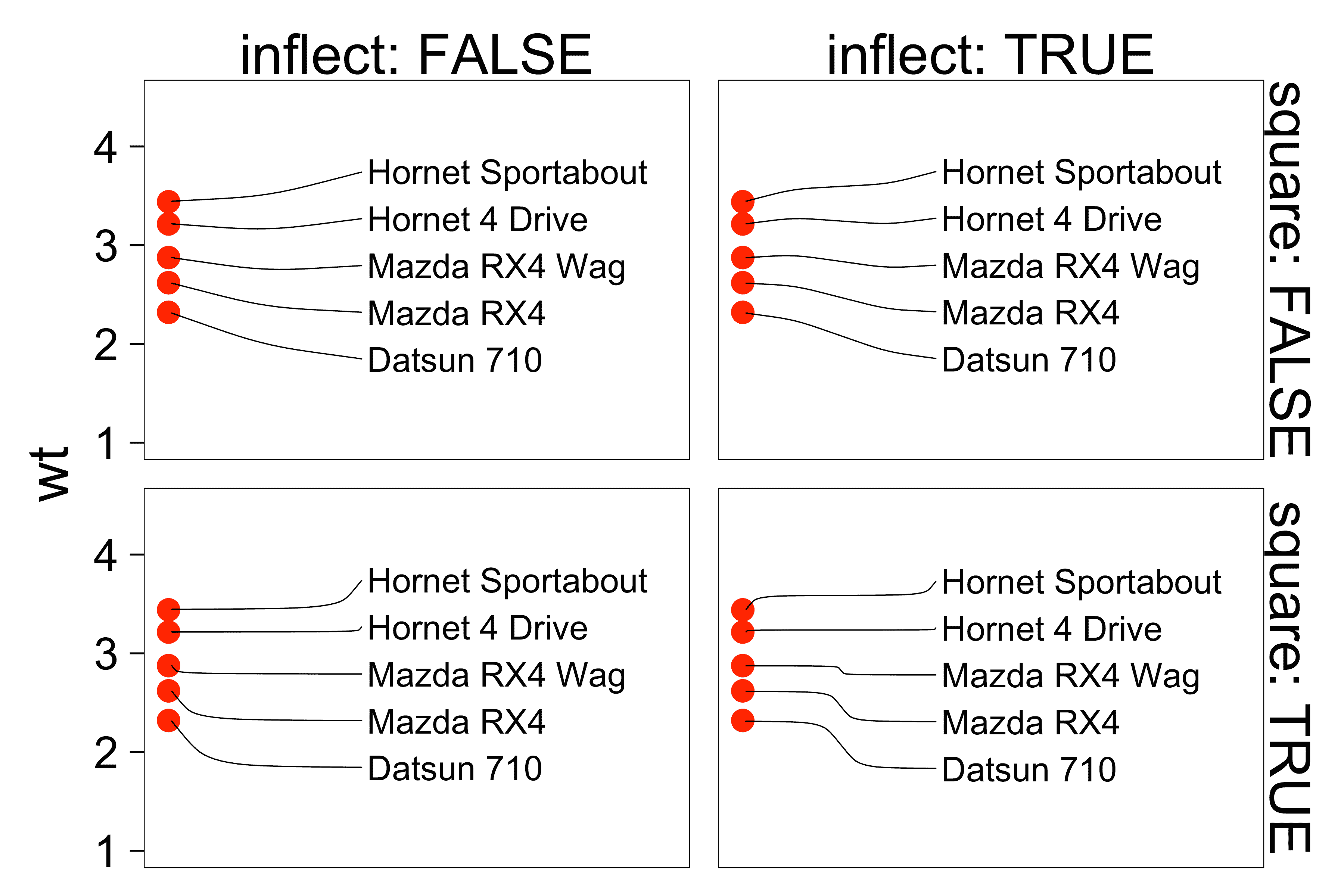Use segment.shape to adjust the interpolation of the control points: set.seed(42) cars_subset_shapes <- cars_subset[rep(seq_len(nrow(cars_subset)), times = 5), ] cars_subset_shapes$shape <- rep(c(-1, -0.5, 0, 0.5, 1), each = nrow(cars_subset))

ggplot(cars_subset_shapes, aes(y = wt, x = 1, label = car)) +
facet_wrap('shape', labeller = labeller(.default = label_both), ncol = 1) +
geom_point(color = "red") +
ylim(1, 4.5) +
xlim(1, 1.375) +
geom_text_repel(
aes(
segment.shape   = shape
),
force             = 0.5,
nudge_x           = 0.25,
direction         = "y",
hjust             = 0,
segment.size      = 0.2,
segment.curvature = -0.6,
segment.angle     = 45,
segment.ncp       = 2,
segment.square    = FALSE,
segment.inflect   = TRUE
) +
theme(
axis.line.x  = element_blank(),
axis.ticks.x = element_blank(),
axis.text.x  = element_blank(),
axis.title.x = element_blank()
)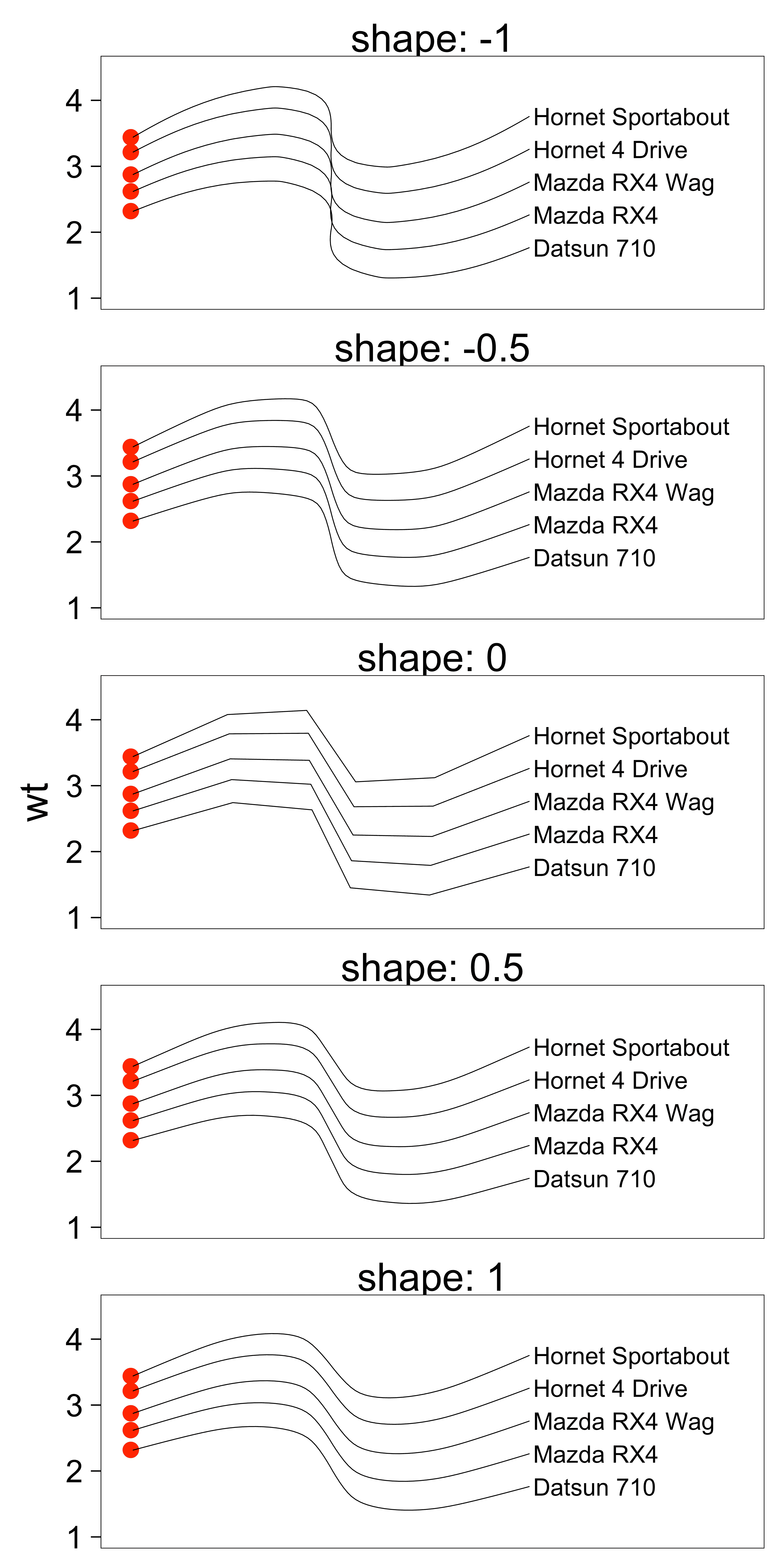We can use different line types (1, 2, 3, 4, 5, or 6).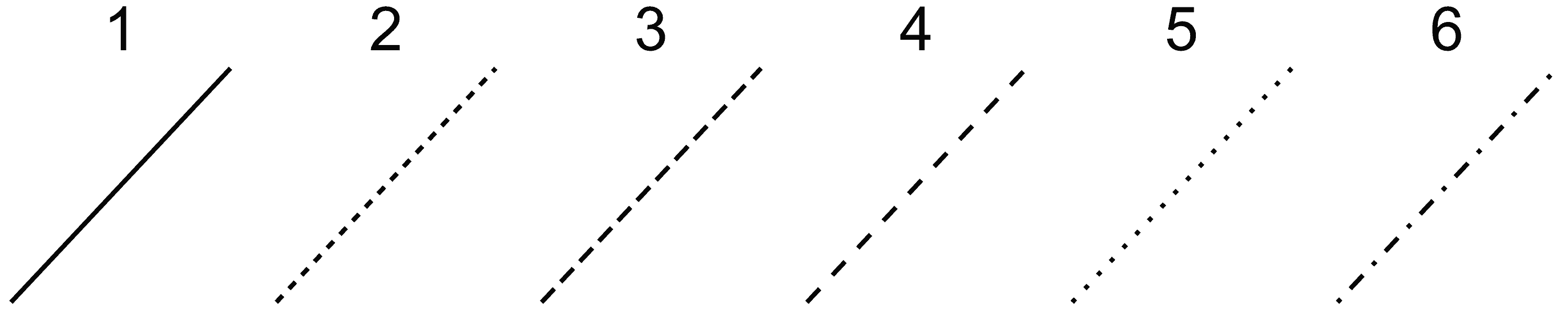And different types of arrows. See ggplot2::geom_segment() for more details.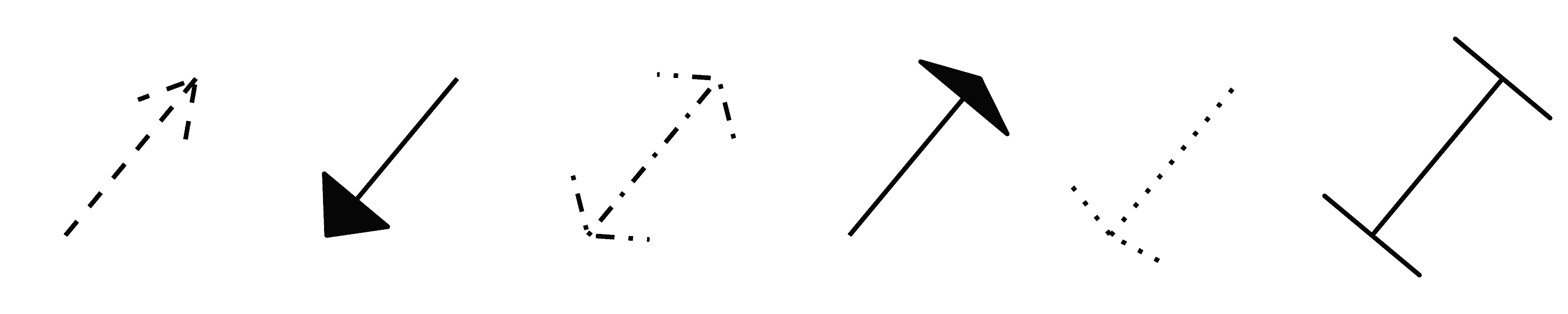set.seed(42)
cars <- c("Volvo 142E", "Merc 230")

ggplot(dat, aes(wt, mpg, label = ifelse(car %in% cars, car, ""))) +
geom_point(color = "red") +
geom_text_repel(
nudge_x = .15,
nudge_y = .5,
segment.linetype = 6,
segment.curvature = -1e-20,
arrow = arrow(length = unit(0.015, "npc"))
)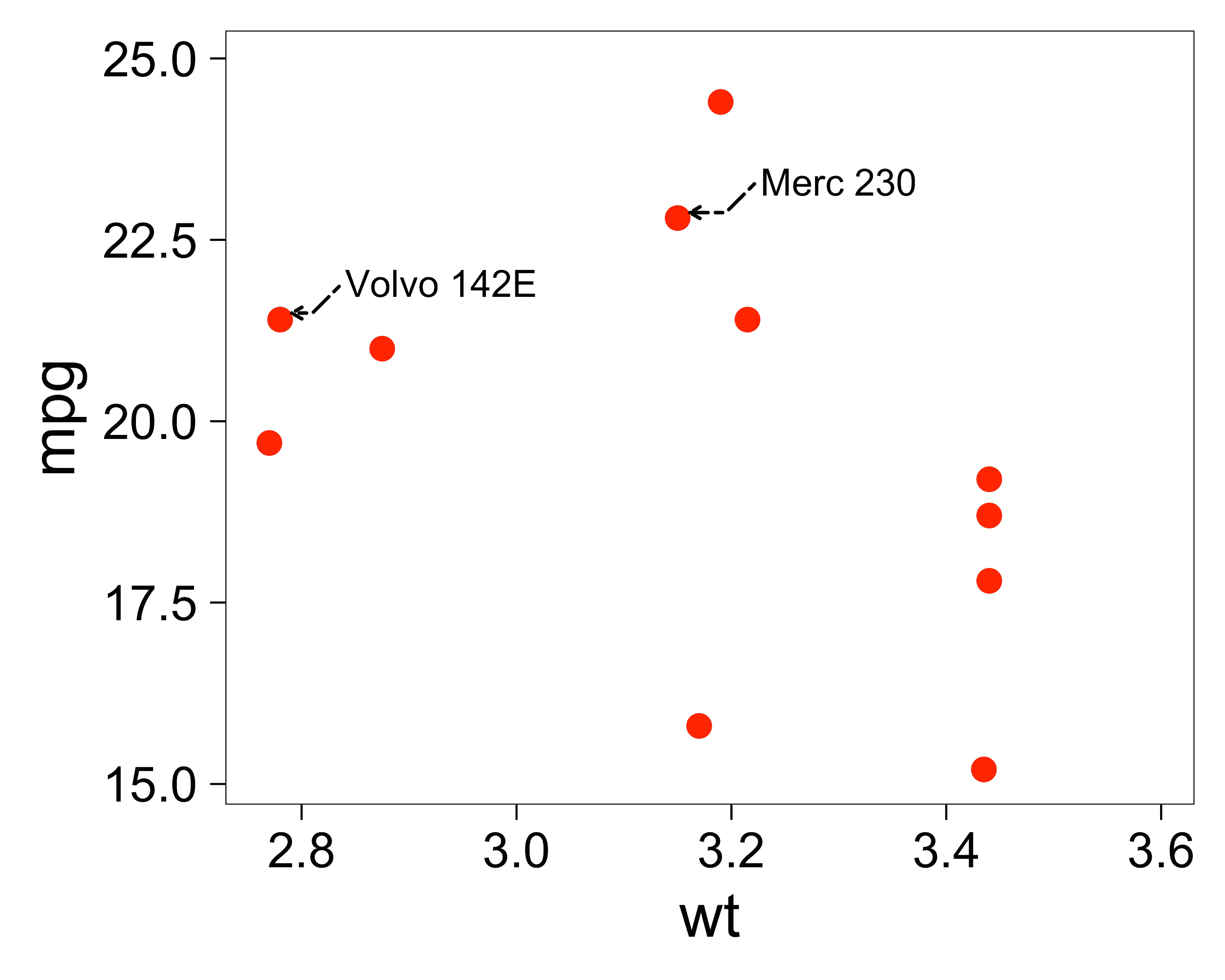### Repel labels from data points with different sizes

We can use the continuous_scale() function from ggplot2. It allows us to specify a single scale that applies to multiple aesthetics.

For ggrepel, we want to apply a single size scale to two aesthetics:

• size, which tells ggplot2 the size of the points to draw on the plot
• point.size, which tells ggrepel the point size, so it can position the text labels away from them

In the example below, there is a third size in the call to geom_text_repel() to specify the font size for the text labels.

my_pal <- function(range = c(1, 6)) {
force(range)
function(x) scales::rescale(x, to = range, from = c(0, 1))
}

ggplot(dat, aes(wt, mpg, label = car)) +
geom_point(aes(size = cyl), alpha = 0.6) + # data point size
continuous_scale(
aesthetics = c("size", "point.size"), scale_name = "size",
palette = my_pal(c(2, 15)),
guide = guide_legend(override.aes = list(label = "")) # hide "a" in legend
) +
geom_text_repel(
aes(point.size = cyl), # data point size
size = 5, # font size in the text labels
min.segment.length = 0, # draw all line segments
max.time = 1, max.iter = 1e5, # stop after 1 second, or after 100,000 iterations
) +
theme(legend.position = "right")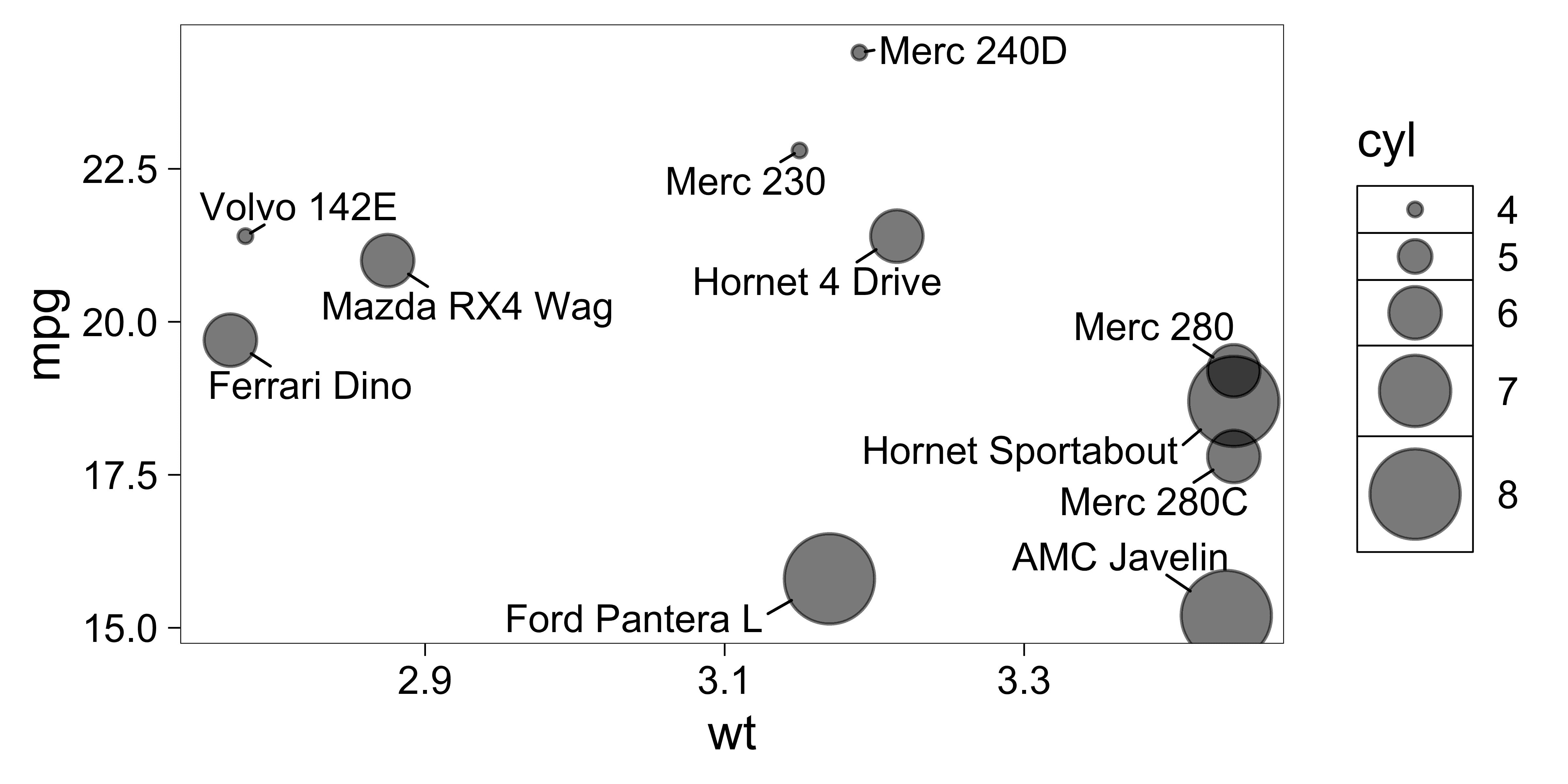my_pal <- function(range = c(1, 6)) {
force(range)
function(x) scales::rescale(x, to = range, from = c(0, 1))
}

ggplot(dat, aes(wt, mpg, label = car)) +
geom_label_repel(
aes(point.size = cyl), # data point size
size = 5, # font size in the text labels
min.segment.length = 0, # draw all line segments
max.time = 1, max.iter = 1e5, # stop after 1 second, or after 100,000 iterations
) +
# Put geom_point() after geom_label_repel, so the
# legend for geom_point() appears on the top layer.
geom_point(aes(size = cyl), alpha = 0.6) + # data point size
continuous_scale(
aesthetics = c("size", "point.size"),
scale_name = "size",
palette = my_pal(c(2, 15)),
guide = guide_legend(override.aes = list(label = "")) # hide "a" in legend
) +
theme(legend.position = "right")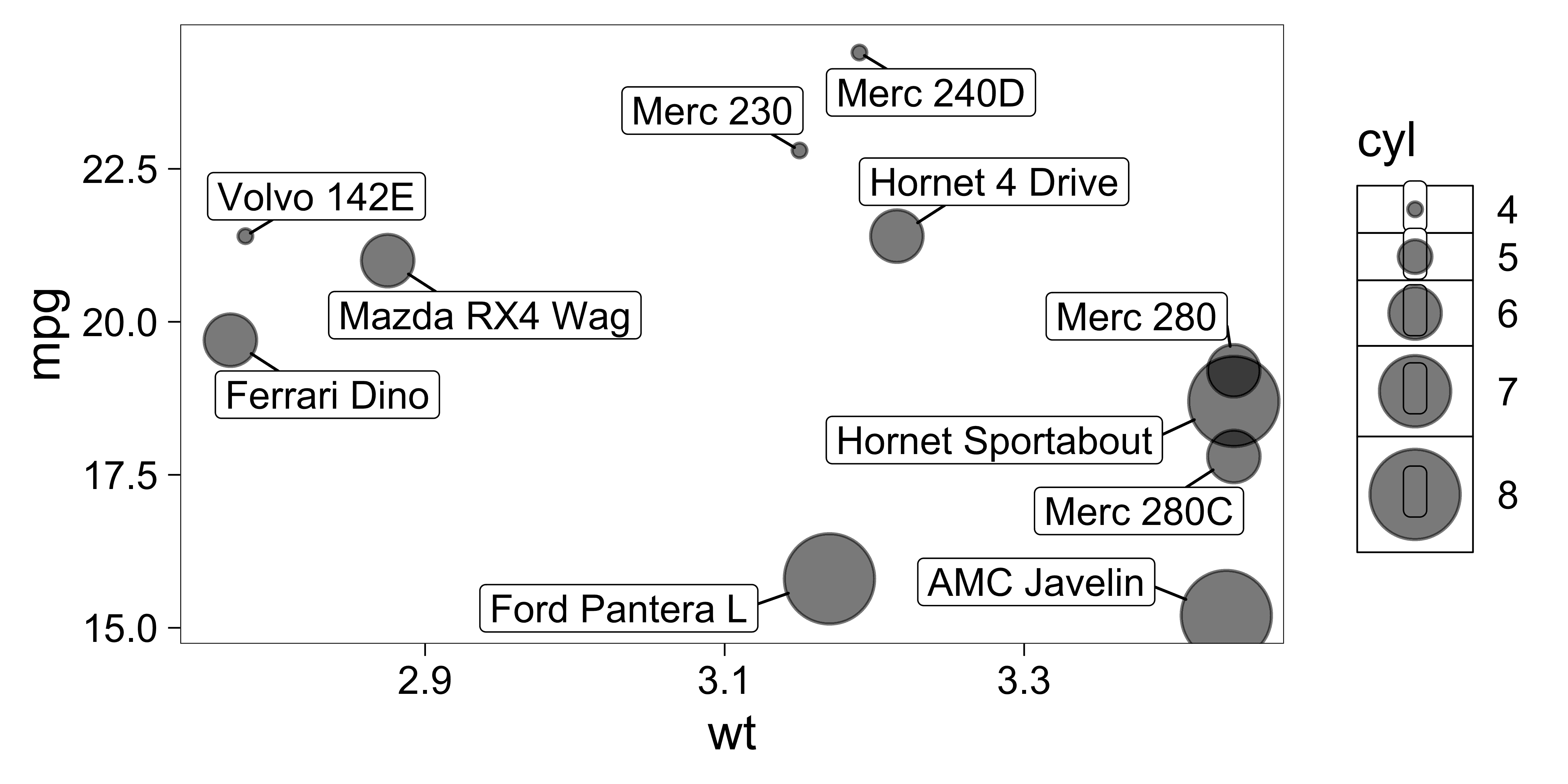### Limit labels to a specific area

Use options xlim and ylim to constrain the labels to a specific area. Limits are specified in data coordinates. Use NA when there is no lower or upper bound in a particular direction.

Here we also use grid::arrow() to render the segments as arrows.

set.seed(42)

# All labels should be to the right of 3.
x_limits <- c(3, NA)

p <- ggplot(dat) +
aes(
x = wt, y = mpg, label = car,
fill = factor(cyl), segment.color = factor(cyl)
) +
geom_vline(xintercept = x_limits, linetype = 3) +
geom_point() +
geom_label_repel(
color = "white",
arrow = arrow(
length = unit(0.03, "npc"), type = "closed", ends = "first"
),
xlim  = x_limits,
) +
scale_fill_discrete(
name = "cyl",
# The same color scall will apply to both of these aesthetics.
aesthetics = c("fill", "segment.color")
)

p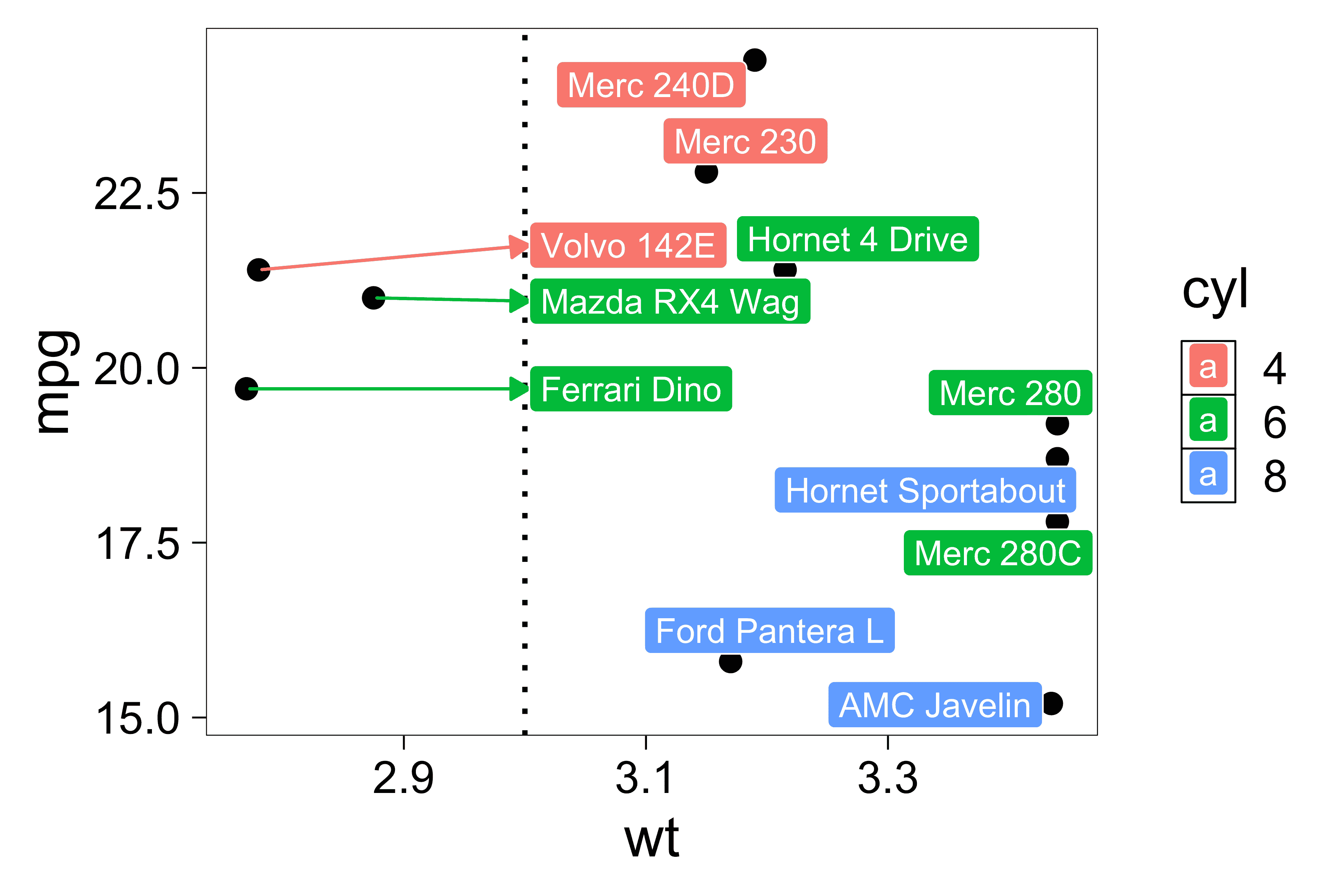### Remove “a” from the legend

Sometimes we want to remove the “a” labels in the legend.

We can do that by overriding the legend aesthetics:

# Don't use "color" in the legend.
p + guides(fill = guide_legend(override.aes = aes(color = NA)))
# Or set the label to the empty string "" (or any other string).
p + guides(fill = guide_legend(override.aes = aes(label = "")))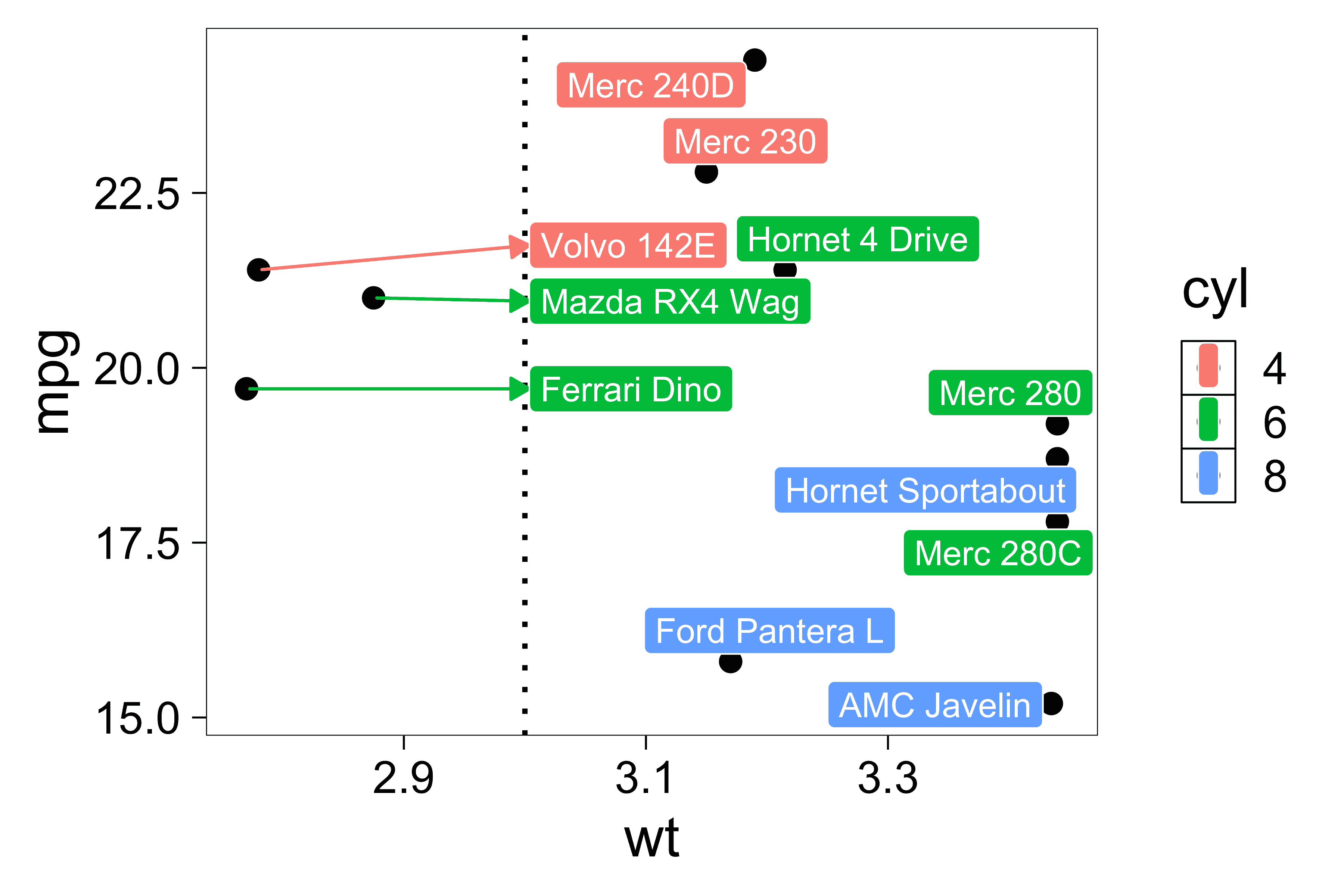### Align labels on the top or bottom edge

Use hjust to justify the text neatly:

• hjust = 0 for left-align
• hjust = 0.5 for center
• hjust = 1 for right-align

Sometimes the labels do not align perfectly. Try using direction = "x" to limit label movement to the x-axis (left and right) or direction = "y" to limit movement to the y-axis (up and down). The default is direction = "both".

Also try using xlim() and ylim() to increase the size of the plotting area so all of the labels fit comfortably.

set.seed(42)

ggplot(mtcars, aes(x = wt, y = 1, label = rownames(mtcars))) +
geom_point(color = "red") +
geom_text_repel(
force_pull   = 0, # do not pull toward data points
nudge_y      = 0.05,
direction    = "x",
angle        = 90,
hjust        = 0,
segment.size = 0.2,
max.iter = 1e4, max.time = 1
) +
xlim(1, 6) +
ylim(1, 0.8) +
theme(
axis.line.y  = element_blank(),
axis.ticks.y = element_blank(),
axis.text.y  = element_blank(),
axis.title.y = element_blank()
)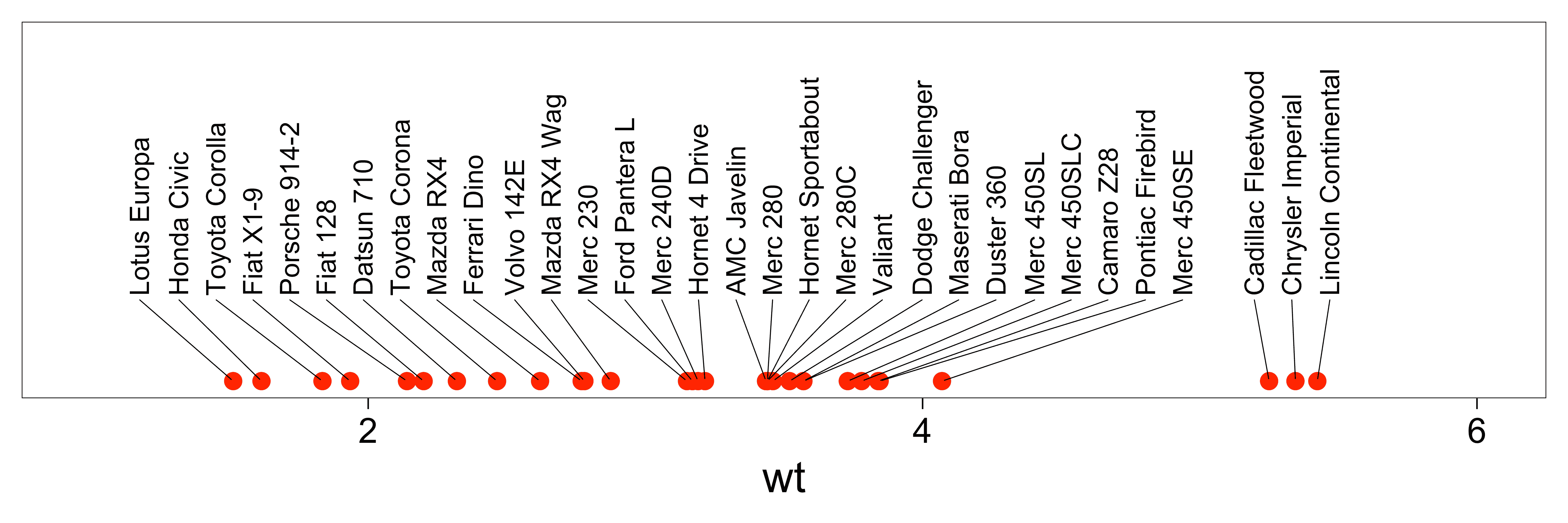Align text vertically with nudge_y and allow the labels to move horizontally with direction = "x":

set.seed(42)

dat <- mtcars
dat$car <- rownames(dat) ggplot(dat, aes(qsec, mpg, label = car)) + geom_text_repel( data = subset(dat, mpg > 30), nudge_y = 36 - subset(dat, mpg > 30)$mpg,
segment.size  = 0.2,
segment.color = "grey50",
direction     = "x"
) +
geom_point(color = ifelse(dat$mpg > 30, "red", "black")) + scale_x_continuous(expand = c(0.05, 0.05)) + scale_y_continuous(limits = c(NA, 36))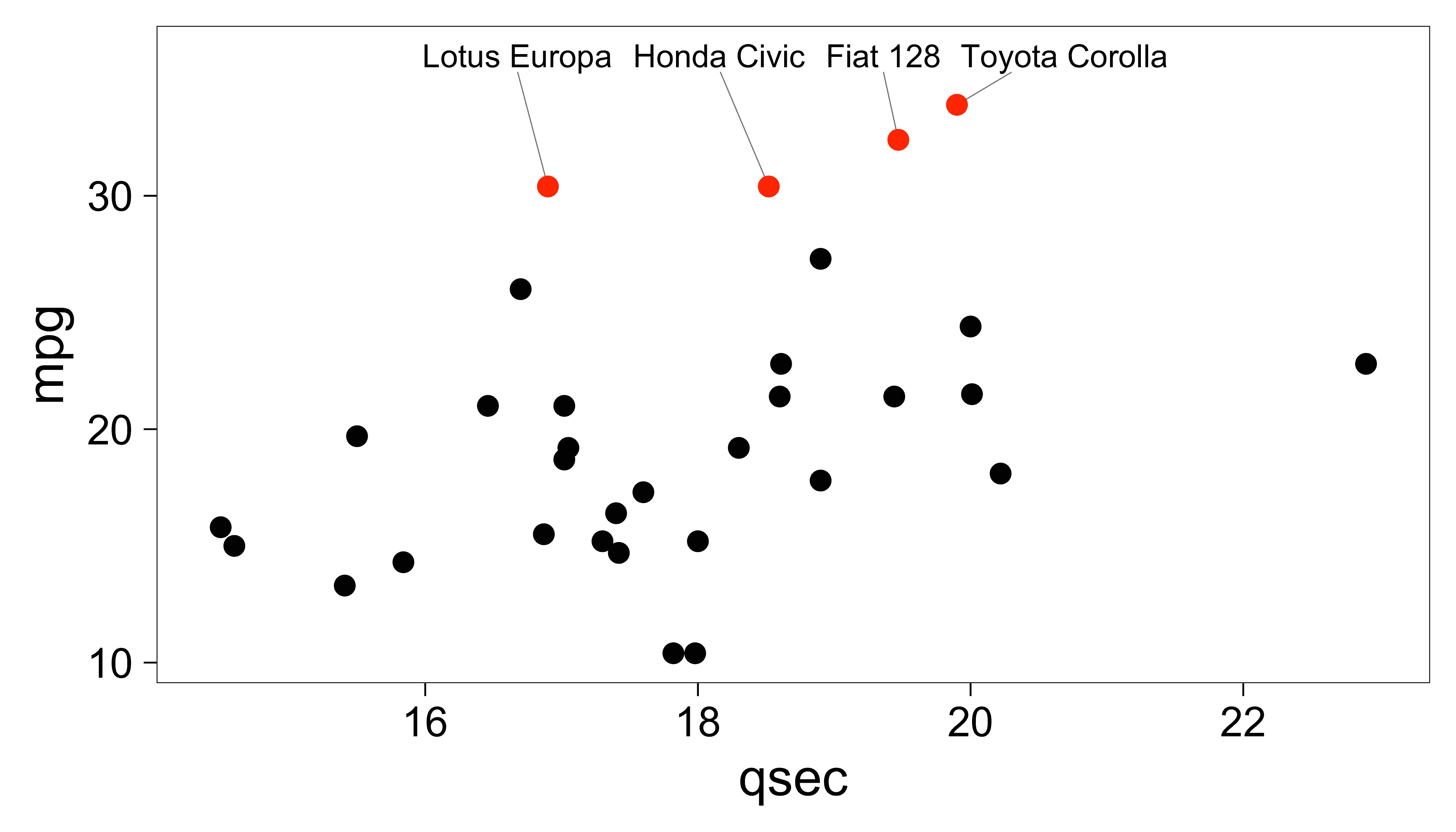### Align labels on the left or right edge Set direction to “y” and try hjust 0.5, 0, and 1: set.seed(42) p <- ggplot(mtcars, aes(y = wt, x = 1, label = rownames(mtcars))) + geom_point(color = "red") + ylim(1, 5.5) + theme( axis.line.x = element_blank(), axis.ticks.x = element_blank(), axis.text.x = element_blank(), axis.title.x = element_blank() ) p1 <- p + xlim(1, 1.375) + geom_text_repel( force = 0.5, nudge_x = 0.15, direction = "y", hjust = 0, segment.size = 0.2 ) + ggtitle("hjust = 0") p2 <- p + xlim(1, 1.375) + geom_text_repel( force = 0.5, nudge_x = 0.2, direction = "y", hjust = 0.5, segment.size = 0.2 ) + ggtitle("hjust = 0.5 (default)") p3 <- p + xlim(0.25, 1) + scale_y_continuous(position = "right") + geom_text_repel( force = 0.5, nudge_x = -0.25, direction = "y", hjust = 1, segment.size = 0.2 ) + ggtitle("hjust = 1") gridExtra::grid.arrange(p1, p2, p3, ncol = 3)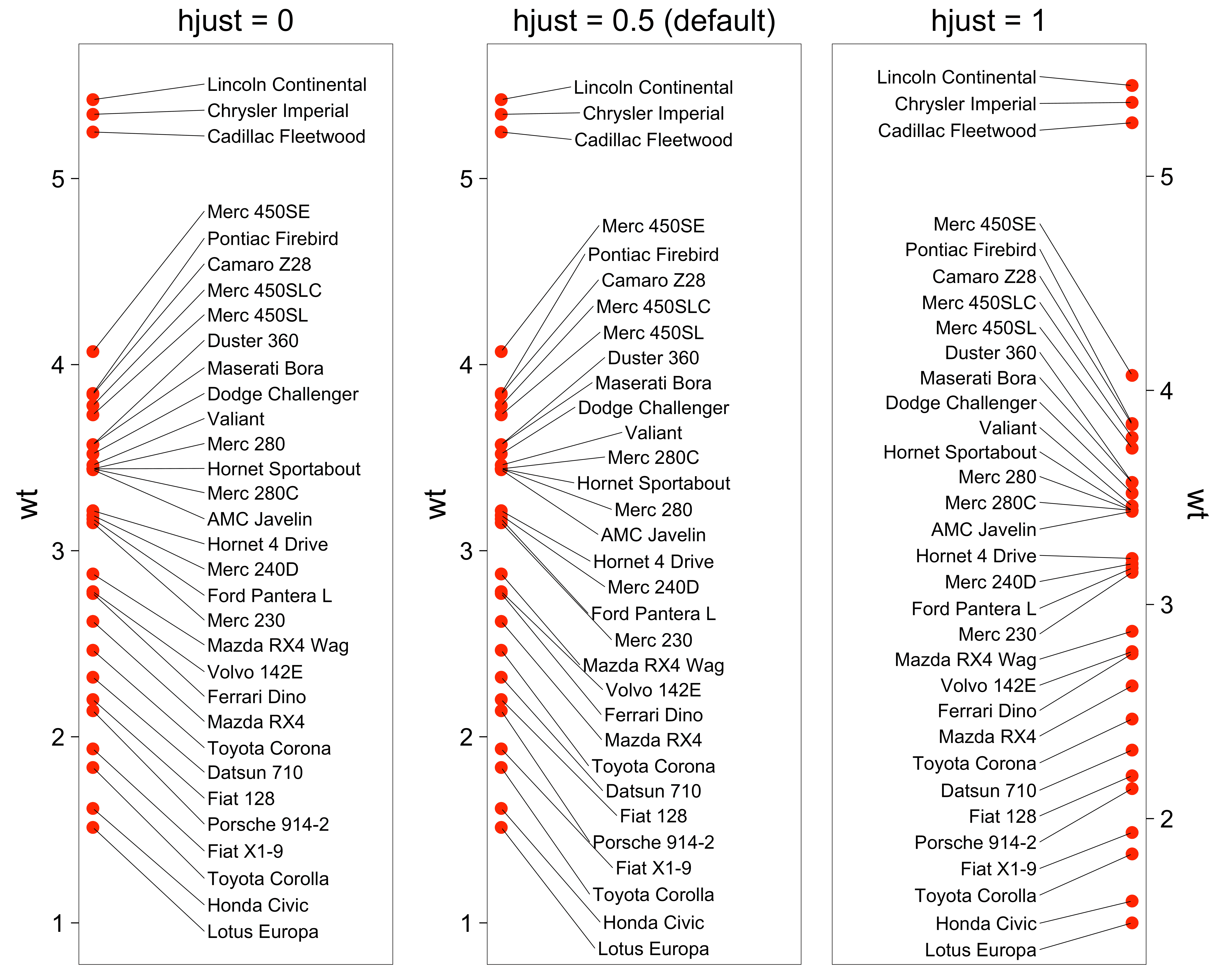Align text horizontally with nudge_x and hjust, and allow the labels to move vertically with direction = "y": set.seed(42) dat <- subset(mtcars, wt > 2.75 & wt < 3.45) dat$car <- rownames(dat)

ggplot(dat, aes(wt, mpg, label = car)) +
geom_text_repel(
data          = subset(dat, wt > 3),
nudge_x       = 3.5 - subset(dat, wt > 3)$wt, segment.size = 0.2, segment.color = "grey50", direction = "y", hjust = 0 ) + geom_text_repel( data = subset(dat, wt < 3), nudge_x = 2.7 - subset(dat, wt < 3)$wt,
segment.size  = 0.2,
segment.color = "grey50",
direction     = "y",
hjust         = 1
) +
scale_x_continuous(
breaks = c(2.5, 2.75, 3, 3.25, 3.5),
limits = c(2.4, 3.8)
) +
geom_point(color = "red")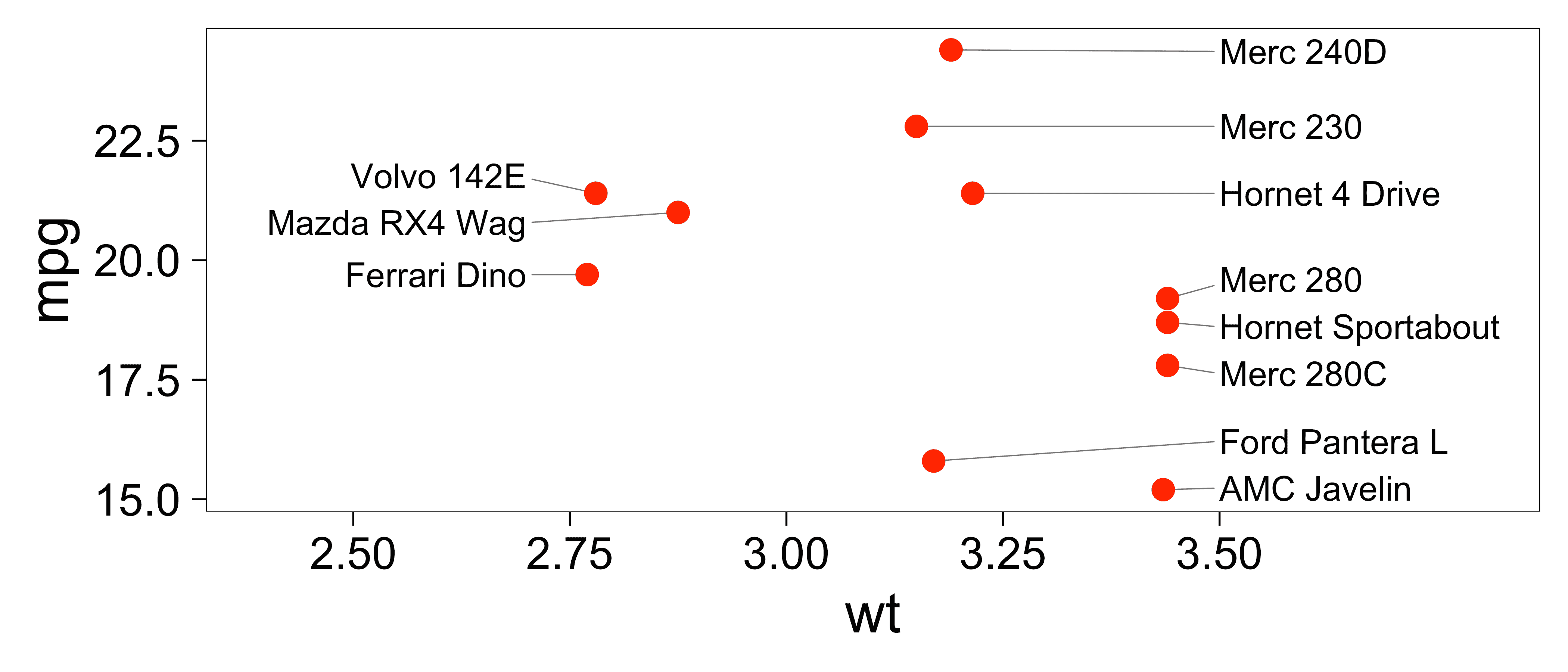### Using ggrepel with stat_summary()

We can use stat_summary() with geom = "text_repel".

The position_nudge_repel() function nudges the text label’s position, but it also remembers the original position of the data point.

p <- ggplot(mtcars, aes(factor(cyl), mpg)) +
stat_summary(
fill = "gray90",
colour = "black",
fun = "mean",
geom = "col"
)

p1 <- p + stat_summary(
aes(label = round(stat(y))),
fun = "mean",
geom = "text_repel",
min.segment.length = 0, # always draw segments
position = position_nudge(y = -2)
) +
labs(title = "position_nudge()")

p2 <- p + stat_summary(
aes(label = round(stat(y))),
fun = "mean",
geom = "text_repel",
min.segment.length = 0, # always draw segments
position = position_nudge_repel(y = -2)
) +
labs(title = "position_nudge_repel()")

gridExtra::grid.arrange(p1, p2, ncol = 2)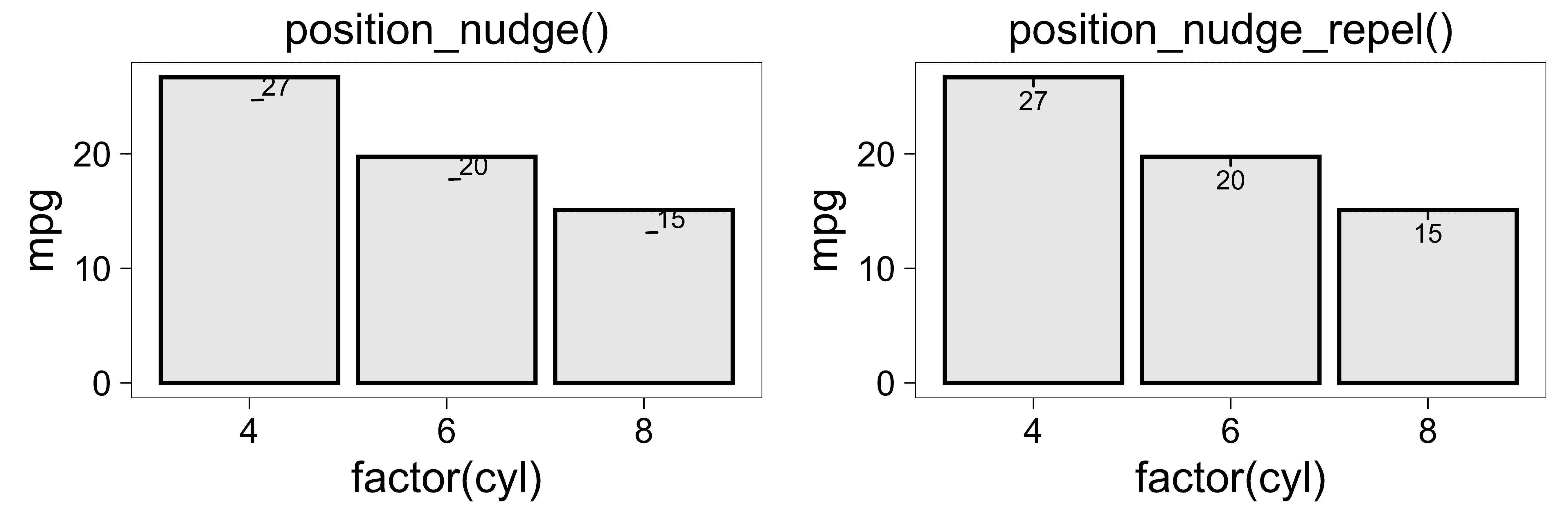### Justify multiple lines of text with hjust

The hjust option should behave mostly the same way it does with ggplot2::geom_text().

p <- ggplot() +
coord_cartesian(xlim=c(0,1), ylim=c(0,1)) +
theme_void()

labelInfo <- data.frame(
x = c(0.45, 0.55),
y = c(0.5, 0.5),
g = c(
"I'd like very much to be\nright justified.",
"And I'd like to be\nleft justified."
)
)

p + geom_text_repel(
data          = labelInfo,
mapping       = aes(x, y, label = g),
size          = 5,
hjust         = c(1, 0),
nudge_x       = c(-0.05, 0.05),
arrow         = arrow(length = unit(2, "mm"), ends = "last", type = "closed")
)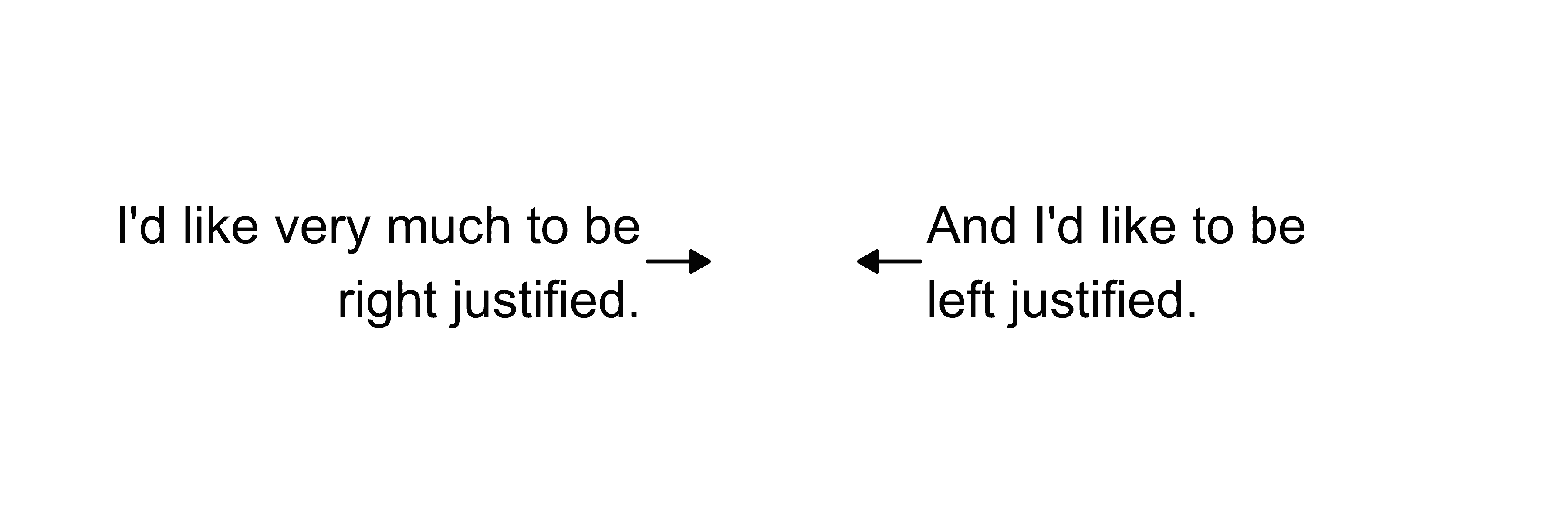p + geom_label_repel(
data          = labelInfo,
mapping       = aes(x, y, label = g),
size          = 5,
hjust         = c(1, 0),
nudge_x       = c(-0.05, 0.05),
arrow         = arrow(length = unit(2, "mm"), ends = "last", type = "closed")
)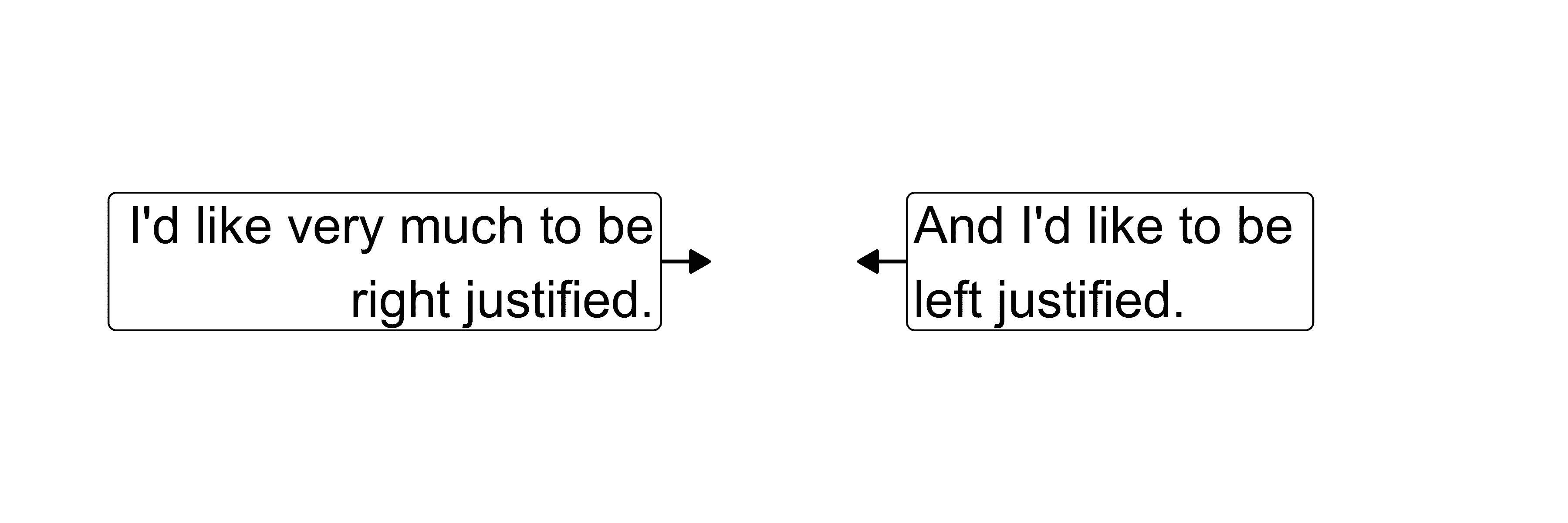### Label jittered points

To get the latest development version of ggplot2, try:

# install.packages("devtools")
devtools::install_github("tidyverse/ggplot2")

mtcars$label <- rownames(mtcars) mtcars$label[mtcars$cyl != 6] <- "" # New! (not available in ggplot2 version 2.2.1 or earlier) pos <- position_jitter(width = 0.3, seed = 2) ggplot(mtcars, aes(factor(cyl), mpg, color = label != "", label = label)) + geom_point(position = pos) + geom_text_repel(position = pos) + theme(legend.position = "none") + labs(title = "position_jitter()")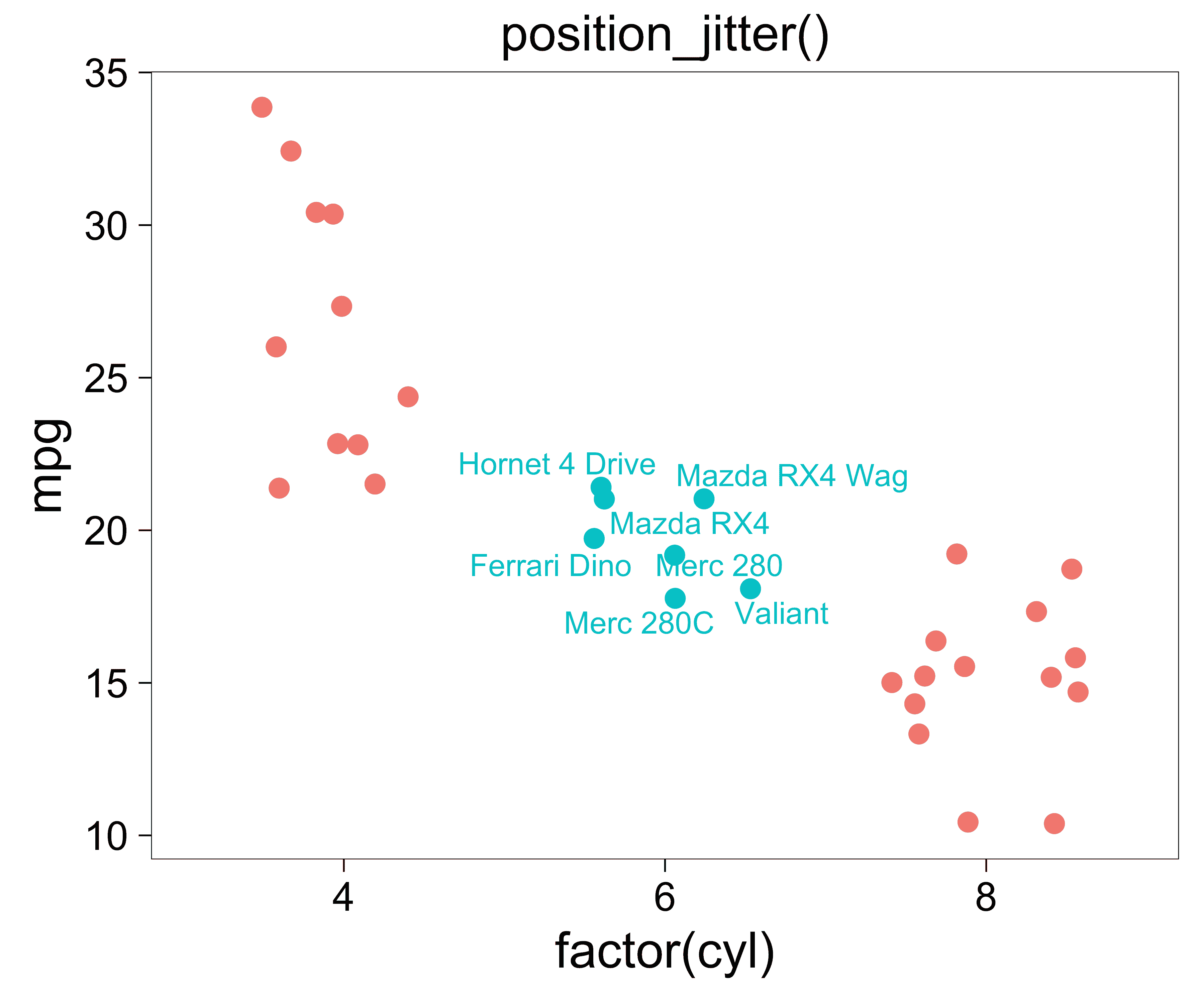You can also use other position functions, like position_quasirandom() from the ggbeeswarm package by Erik Clarke: mtcars$label <- rownames(mtcars)
mtcars$label[mtcars$cyl != 6] <- ""

library(ggbeeswarm)
pos <- position_quasirandom()

ggplot(mtcars, aes(factor(cyl), mpg, color = label != "", label = label)) +
geom_point(position = pos) +
geom_text_repel(position = pos) +
theme(legend.position = "none") +
labs(title = "position_quasirandom()")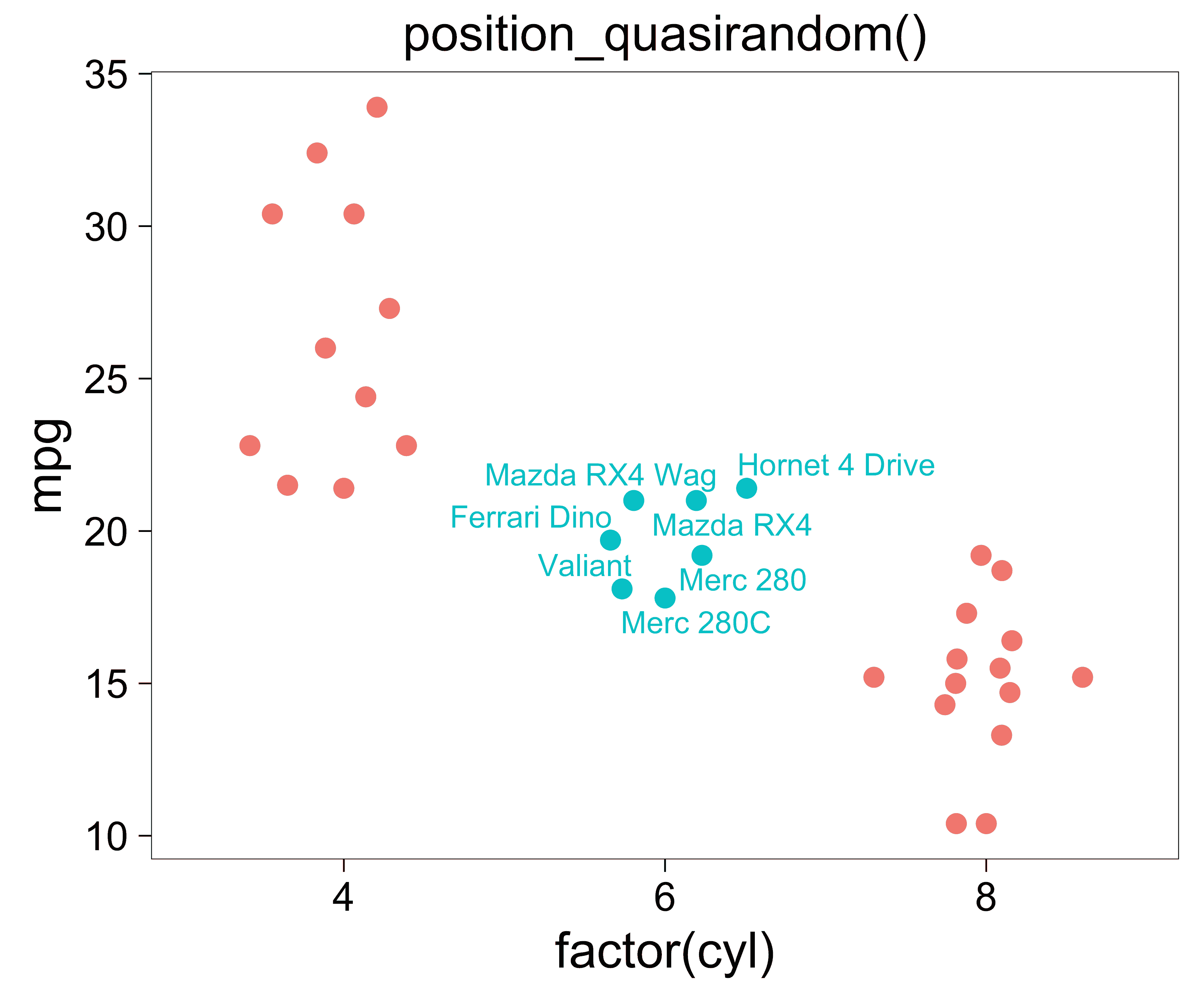### Label sf objects

Currently if you use geom_text_repel() or geom_label_repel() with a ggplot2::geom_sf plot, you will probably get an error like

Error: geom_label_repel requires the following missing aesthetics: x and y

There’s a workaround to this which will enable the ggrepel functions to work with spatial sf plots like this - you just need to include:

stat = "sf_coordinates"

in the geom_text|label_repel() call.

# thanks to Hiroaki Yutani
# https://github.com/slowkow/ggrepel/issues/111#issuecomment-416853013

library(ggplot2)
library(sf)

nc <- sf::st_read(system.file("shape/nc.shp", package="sf"), quiet = TRUE)

ggplot(nc) +
geom_sf() +
ggrepel::geom_label_repel(
aes(label = NAME, geometry = geometry),
stat = "sf_coordinates",
min.segment.length = 0
)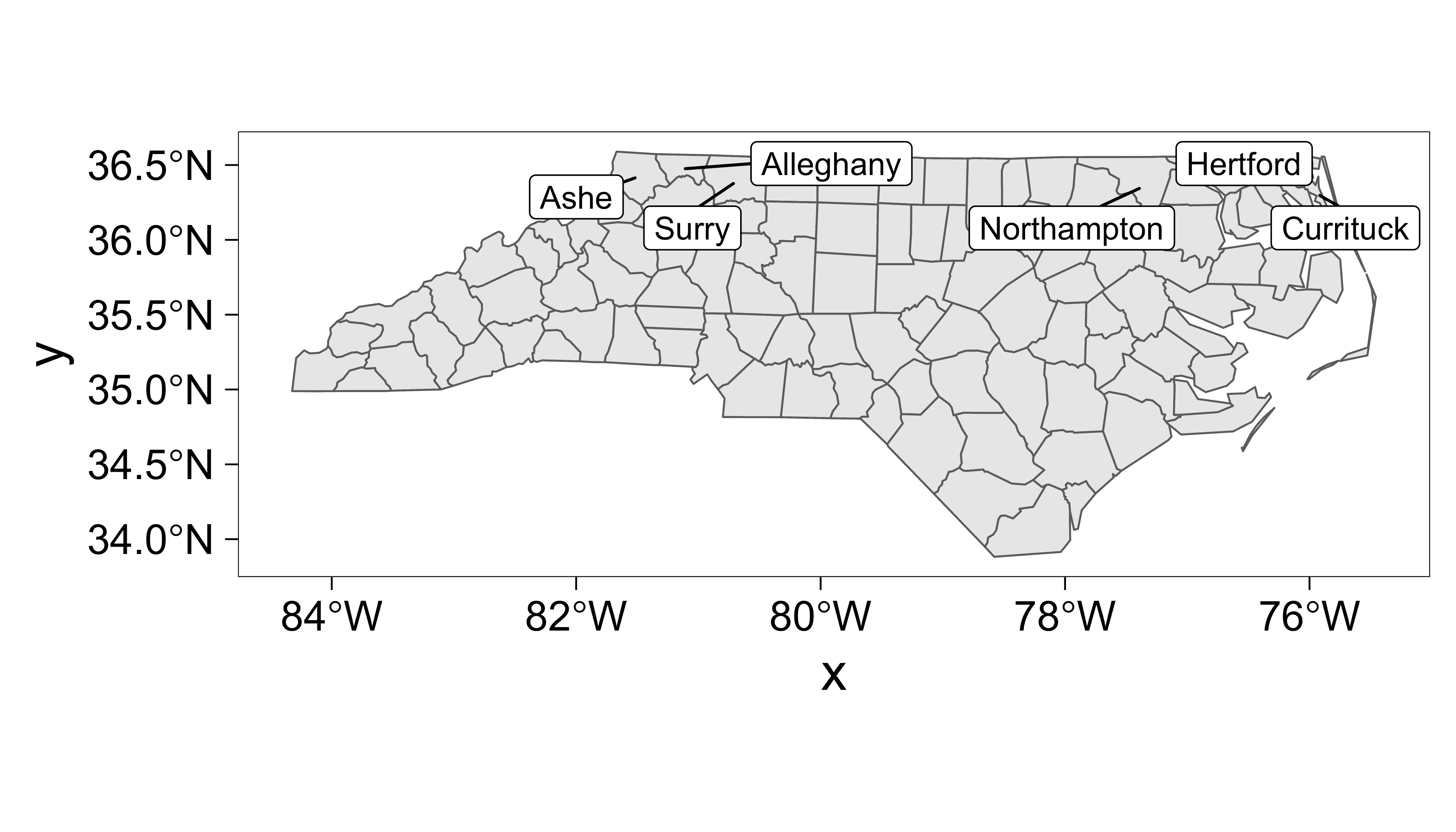Thanks to Hiroaki Yutani for the solution.

### Shadows (or glow) under text labels

We can place shadows (or glow) underneath each text label to enhance the readability of the text. This might be useful when text labels are placed on top of other plot elements. This feature uses the same code as the shadowtext package by Guangchuang Yu.

set.seed(42)
ggplot(dat, aes(wt, mpg, label = car)) +
geom_point(color = "red") +
geom_text_repel(
color = "white",     # text color
bg.color = "grey30", # shadow color
)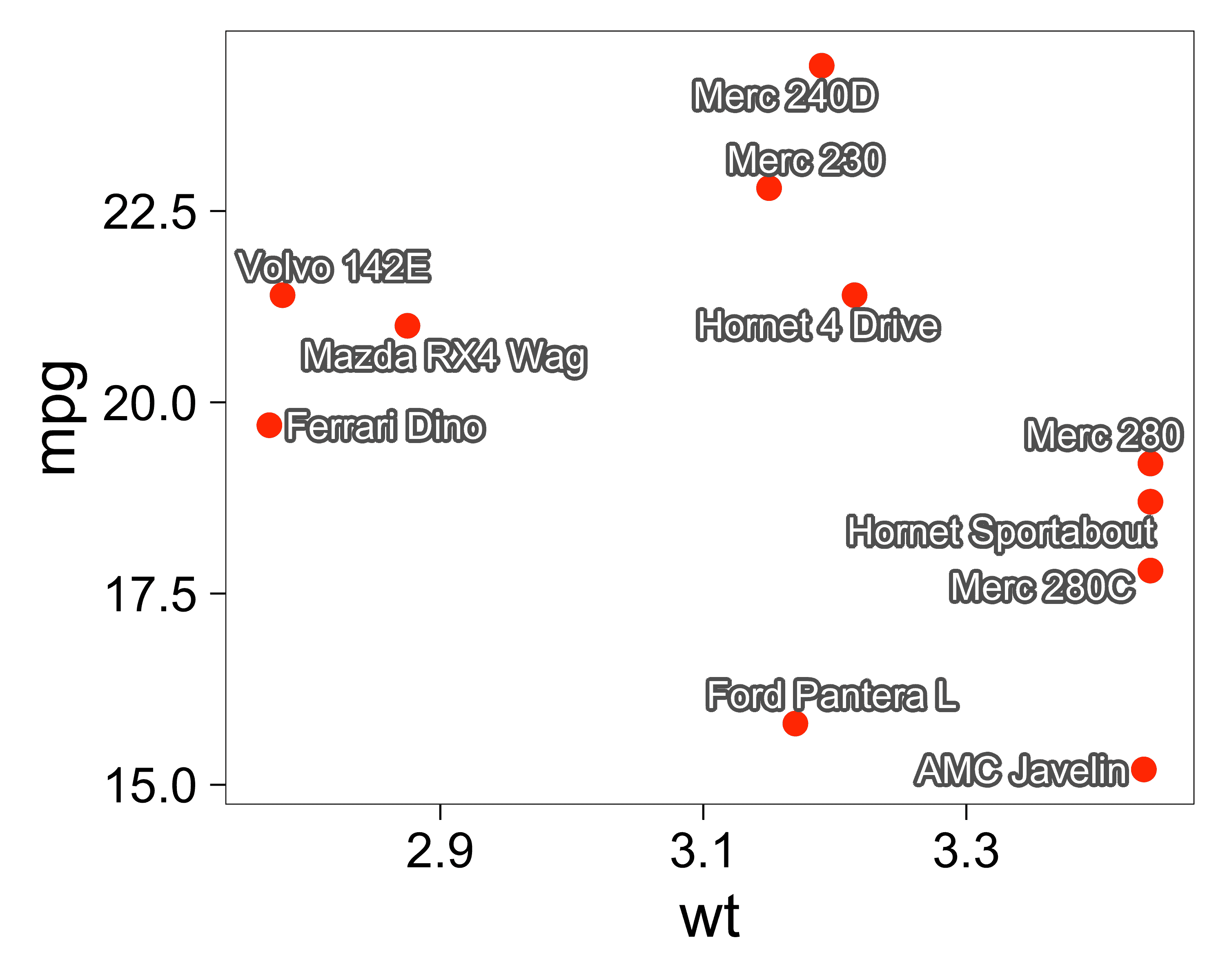### Verbose timing information

Use verbose = TRUE to see:

• how many iterations of the physical simulation were completed
• how much time has elapsed, in seconds
• how many overlaps remain unresolved in the final figure
p <- ggplot(mtcars,
aes(wt, mpg, label = rownames(mtcars), colour = factor(cyl))) +
geom_point()

p + geom_text_repel(
verbose = TRUE,
seed = 123,
max.time = 1,
max.iter = Inf,
size = 3
)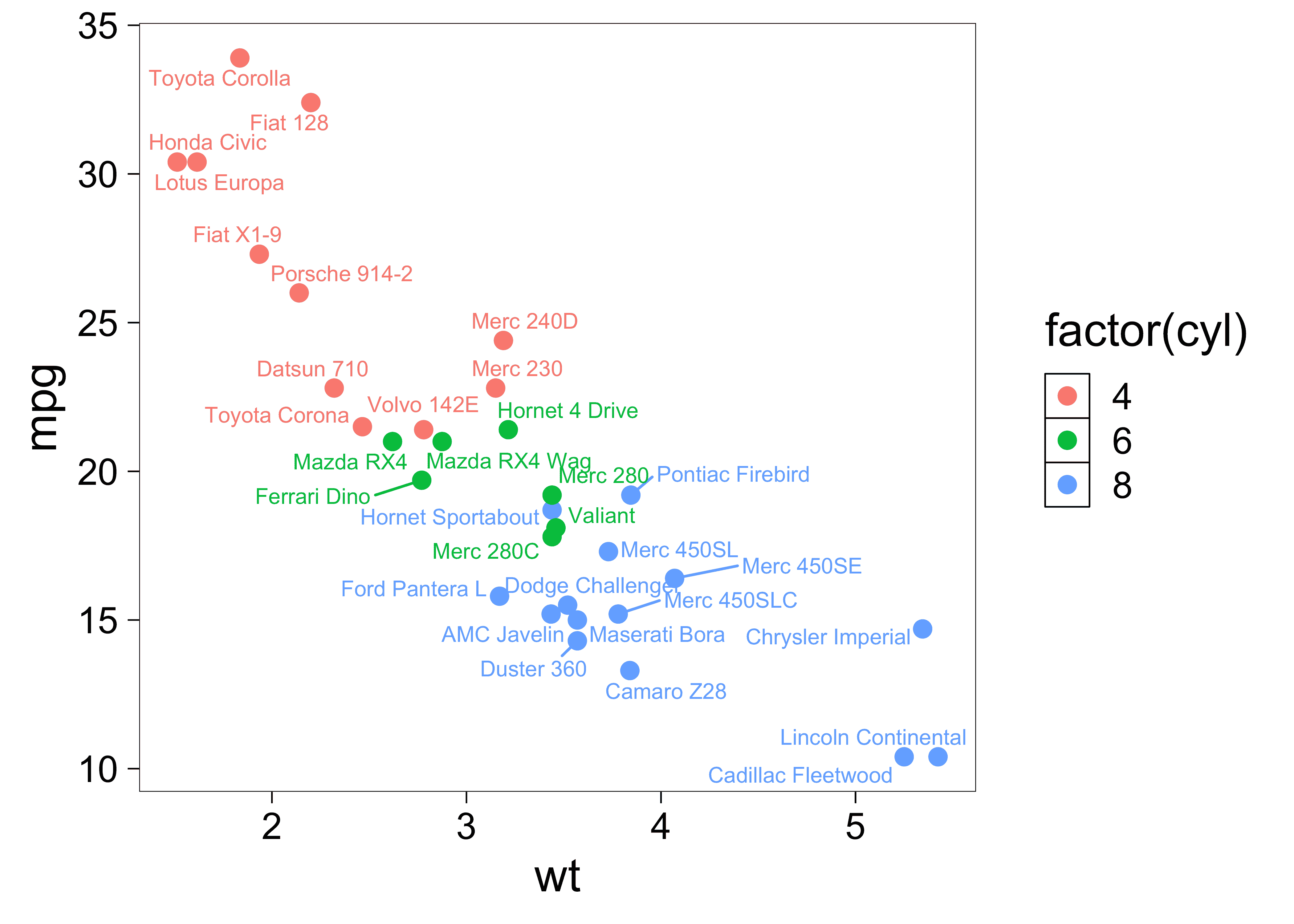## 1.00s elapsed for 163540 iterations, 2 overlaps. Consider increasing 'max.time'.

### Word cloud

The force option controls the strength of repulsion.

The force_pull option controls the strength of the spring that pulls the text label toward its data point.

To make a word cloud, we can assign all of the text labels the same data point at the origin (0, 0) and set force_pull = 0 to disable the springs.

set.seed(42)
ggplot(mtcars) +
geom_text_repel(
aes(
label  = rownames(mtcars),
size   = mpg > 15,
colour = factor(cyl),
x      = 0,
y      = 0
),
force_pull    = 0, # do not pull text toward the point at (0,0)
max.time      = 0.5,
max.iter      = 1e5,
max.overlaps  = Inf,
segment.color = NA,
) +
theme_void() +
theme(strip.text = element_text(size = 16)) +
facet_wrap(~ factor(cyl)) +
scale_color_discrete(name = "Cylinders") +
scale_size_manual(values = c(2, 3)) +
theme(
strip.text   = element_blank(),
panel.border = element_rect(size = 0.2, fill = NA)
)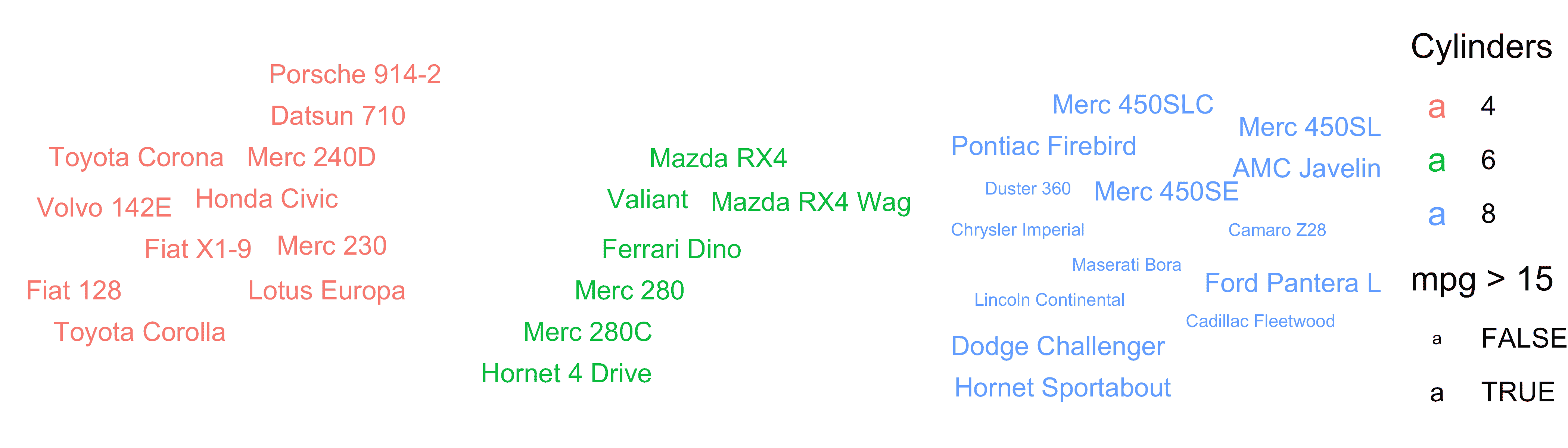### Polar coordinates

set.seed(42)

mtcars$label <- rownames(mtcars) mtcars$label[mtcars\$mpg < 25] <- ""

ggplot(mtcars, aes(x = wt, y = mpg, color = factor(cyl), label = label)) +
coord_polar(theta = "x") +
geom_point(size = 2) +
scale_color_discrete(name = "cyl") +
geom_text_repel(show.legend = FALSE) + # Don't display "a" in the legend.
theme_bw(base_size = 18)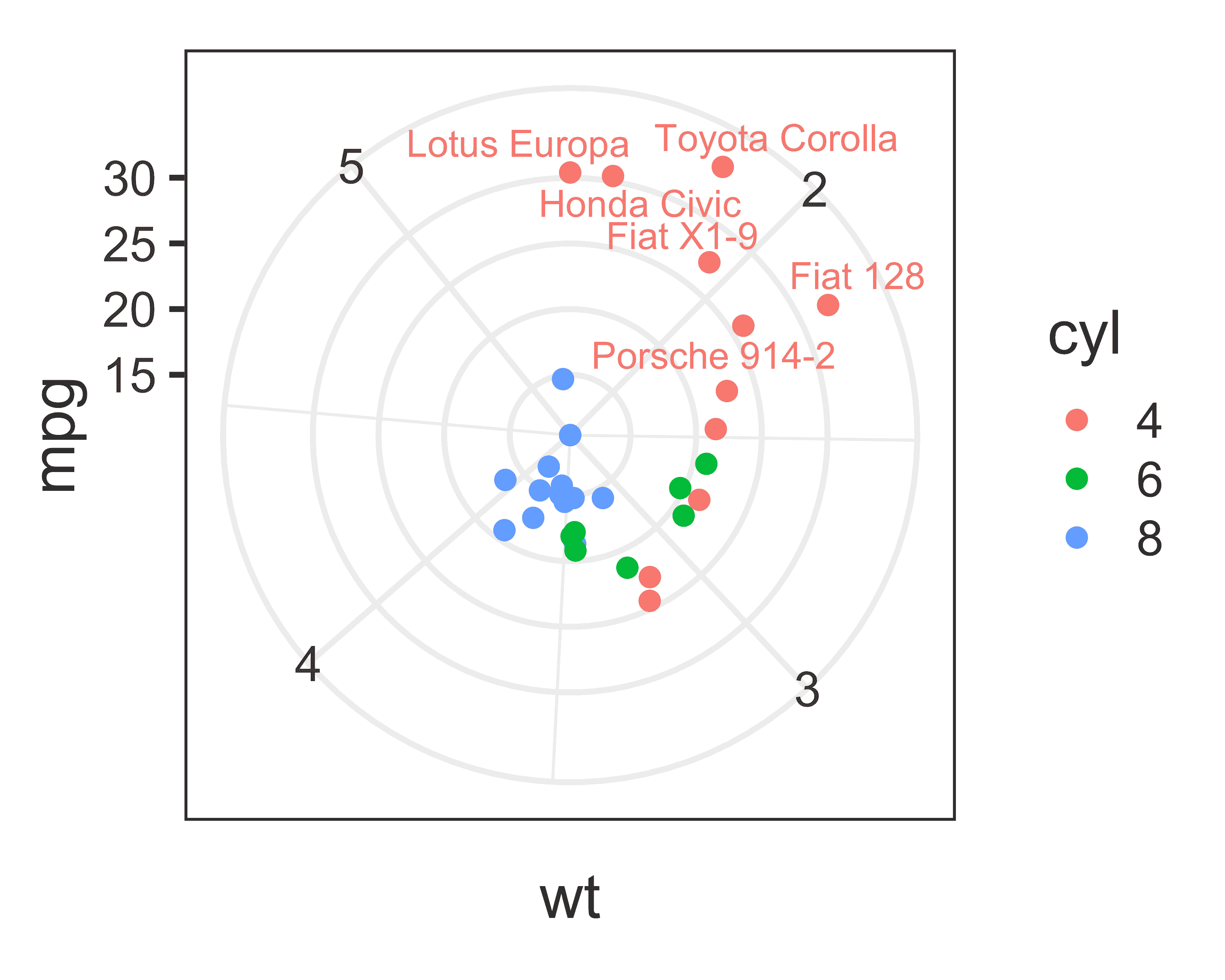### Unicode characters (Japanese)

library(ggrepel)

set.seed(42)
dat <- data.frame(
x = runif(32),
y = runif(32),
label = strsplit(
x = "原文篭毛與美篭母乳布久思毛與美夫君志持此岳尓菜採須兒家吉閑名思毛",
split = ""
)[]
)

# Make sure to choose a font that is installed on your system.
my_font <- "HiraginoSans-W0"

ggplot(dat, aes(x, y, label = label)) +
geom_point(size = 2, color = "red") +
geom_text_repel(size = 8, family = my_font) +
ggtitle("テスト") +
theme_bw(base_size = 18, base_family = my_font)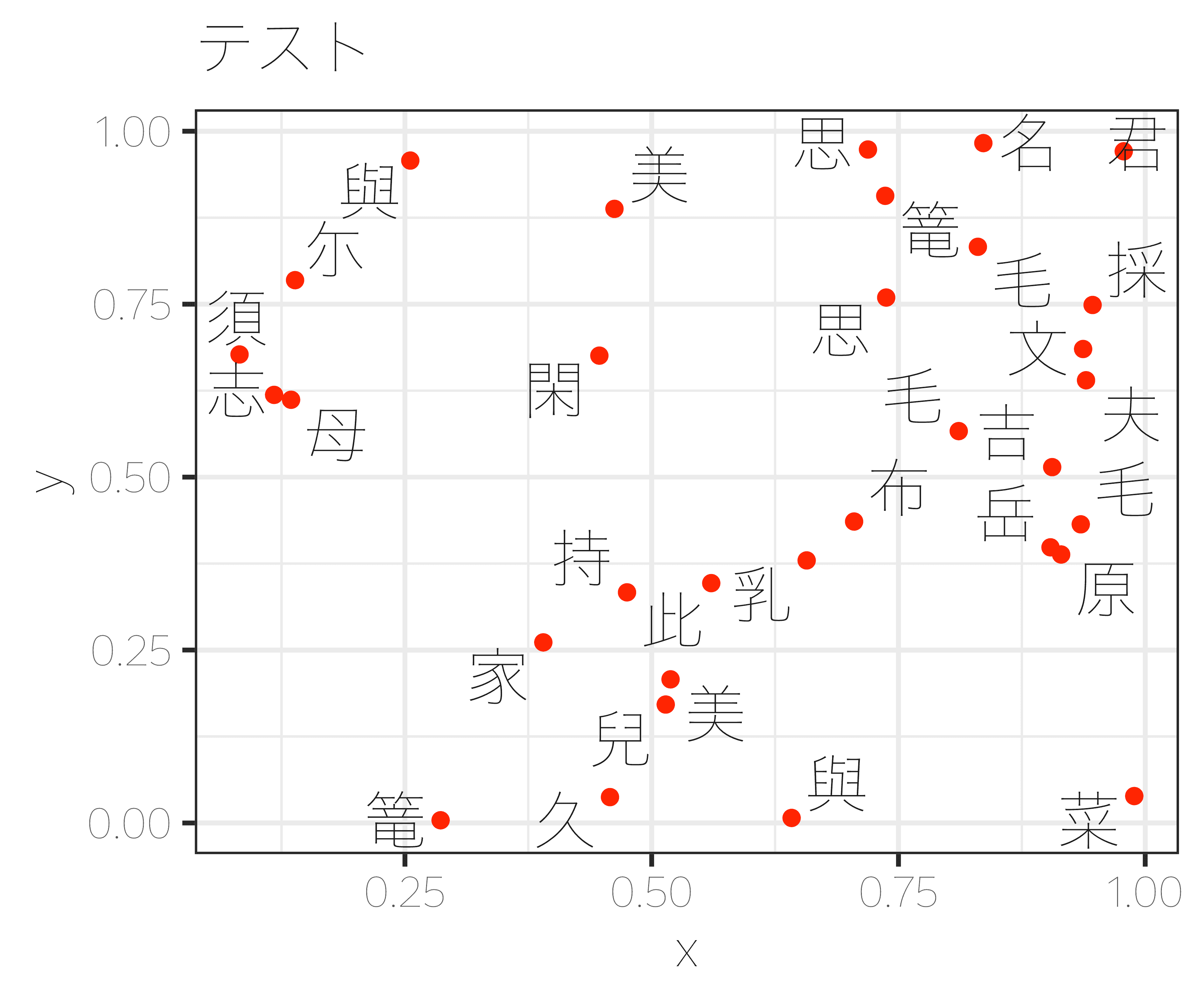### Mathematical expressions

d <- data.frame(
x    = c(1, 2, 2, 1.75, 1.25),
y    = c(1, 3, 1, 2.65, 1.25),
math = c(
NA,
"integral(f(x) * dx, a, b)",
NA,
"lim(f(x), x %->% 0)",
NA
)
)

ggplot(d, aes(x, y, label = math)) +
geom_point() +
geom_label_repel(
parse       = TRUE, # Parse mathematical expressions.
size        = 6,
)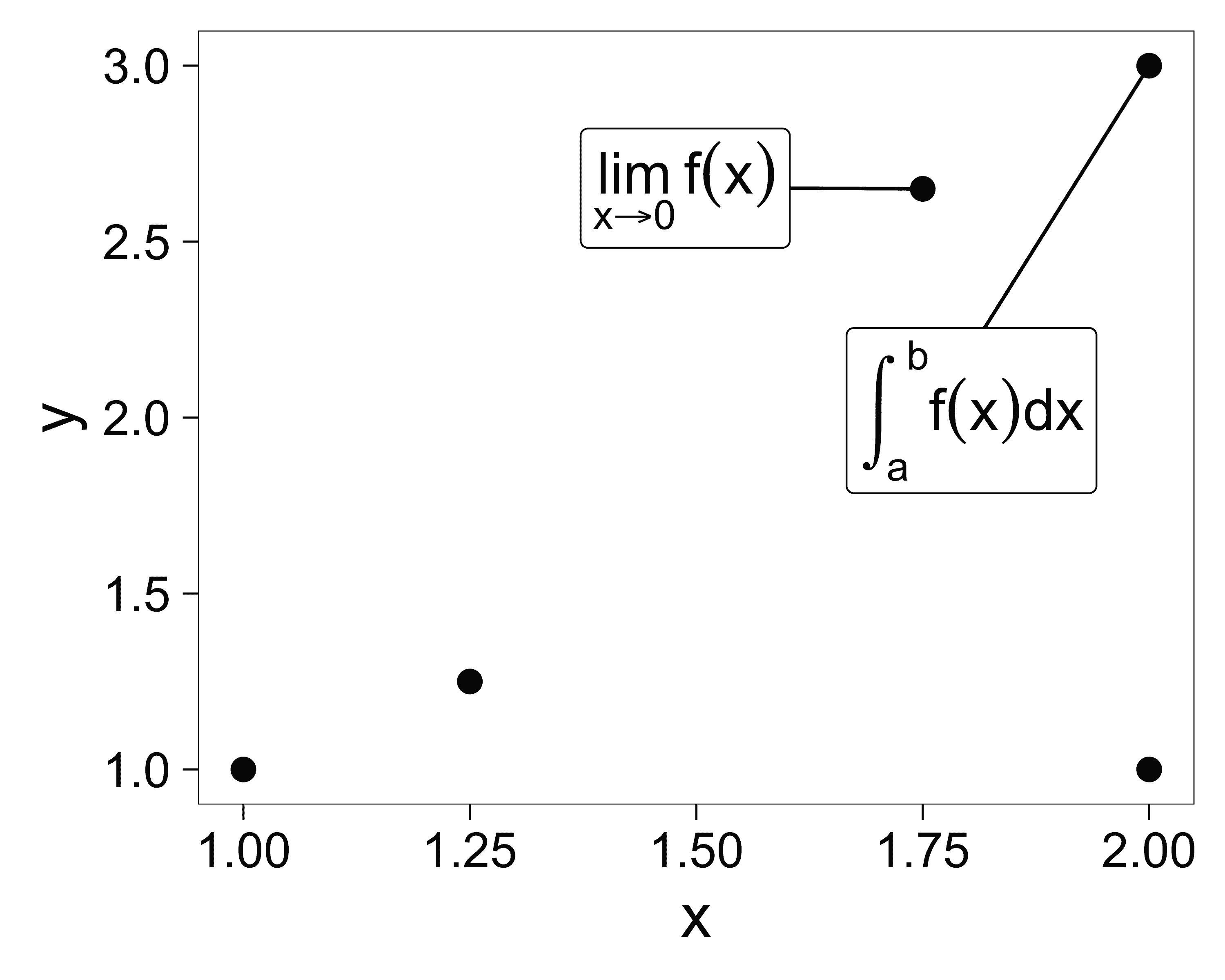### Animation

# This chunk of code will take a minute or two to run.
library(ggrepel)
library(animation)

plot_frame <- function(n) {
set.seed(42)
p <- ggplot(mtcars, aes(wt, mpg, label = rownames(mtcars))) +
geom_text_repel(
size = 5, force = 1, max.iter = n
) +
geom_point(color = "red") +
# theme_minimal(base_size = 16) +
labs(title = n)
print(p)
}

xs <- ceiling(1.18^(1:52))
# xs <- ceiling(1.4^(1:26))
xs <- c(xs, rep(xs[length(xs)], 15))
# plot(xs)

saveGIF(
lapply(xs, function(i) {
plot_frame(i)
}),
interval   = 0.15,
ani.width  = 800,
ani.heigth = 600,
movie.name = "animated.gif"
)

## Source code

View the source code for this vignette on GitHub.

## R Session Info

sessionInfo()
## R version 4.2.3 (2023-03-15)
## Platform: aarch64-apple-darwin20 (64-bit)
## Running under: macOS Ventura 13.2.1
##
## Matrix products: default
## BLAS:   /Library/Frameworks/R.framework/Versions/4.2-arm64/Resources/lib/libRblas.0.dylib
## LAPACK: /Library/Frameworks/R.framework/Versions/4.2-arm64/Resources/lib/libRlapack.dylib
##
## locale:
##  en_US.UTF-8/en_US.UTF-8/en_US.UTF-8/C/en_US.UTF-8/en_US.UTF-8
##
## attached base packages:
##  stats     graphics  grDevices utils     datasets  methods   base
##
## other attached packages:
##  glue_1.6.2       rvest_1.0.3      sf_1.0-12        ggbeeswarm_0.7.2
##  ggrepel_0.9.3    ggplot2_3.4.2    gridExtra_2.3    knitr_1.42
##
## loaded via a namespace (and not attached):
##   beeswarm_0.4.0     tidyselect_1.2.0   xfun_0.39          bslib_0.4.2
##   purrr_1.0.1        colorspace_2.1-0   vctrs_0.6.2        generics_0.1.3
##   htmltools_0.5.5    yaml_2.3.7         utf8_1.2.3         rlang_1.1.1
##  e1071_1.7-13       pkgdown_2.0.7      jquerylib_0.1.4    pillar_1.9.0
##  withr_2.5.0        DBI_1.1.3          lifecycle_1.0.3    stringr_1.5.0
##  munsell_0.5.0      gtable_0.3.3       ragg_1.2.5         codetools_0.2-19
##  memoise_2.0.1      evaluate_0.20      labeling_0.4.2     fastmap_1.1.1
##  vipor_0.4.5        class_7.3-21       fansi_1.0.4        highr_0.10
##  Rcpp_1.0.10        KernSmooth_2.23-20 scales_1.2.1       classInt_0.4-9
##  cachem_1.0.7       desc_1.4.2         jsonlite_1.8.4     farver_2.1.1
##  systemfonts_1.0.4  fs_1.6.2           textshaping_0.3.6  digest_0.6.31
##  stringi_1.7.12     dplyr_1.1.2        grid_4.2.3         rprojroot_2.0.3
##  cli_3.6.1          tools_4.2.3        magrittr_2.0.3     sass_0.4.5
##  proxy_0.4-27       tibble_3.2.1       pkgconfig_2.0.3    xml2_1.3.4
##  httr_1.4.5         rmarkdown_2.21     R6_2.5.1           units_0.8-2
##  compiler_4.2.3